# West Bengal Madhyamik Board Question Paper for Class 10th Maths 2016 In PDF

West Bengal (Class 10) Maths 2016 question paper with solutions are uploaded on this page by BYJU’S in downloadable pdf format and also in the text so that the students can make use of them. Maths Question Paper 2016 Class 10 is added here, which contains all the important concepts that have to be studied for the exams. West Bengal (Class 10) Maths 2016 question paper gives an overview of the question types that are repeatedly asked for the board exams.

Students are able to access all the West Bengal board previous year Maths question papers. Students can assess their confidence level for the upcoming board exams and also score well by practising these papers.

### WBBSE Class 10th Maths Question Paper With Solutions 2016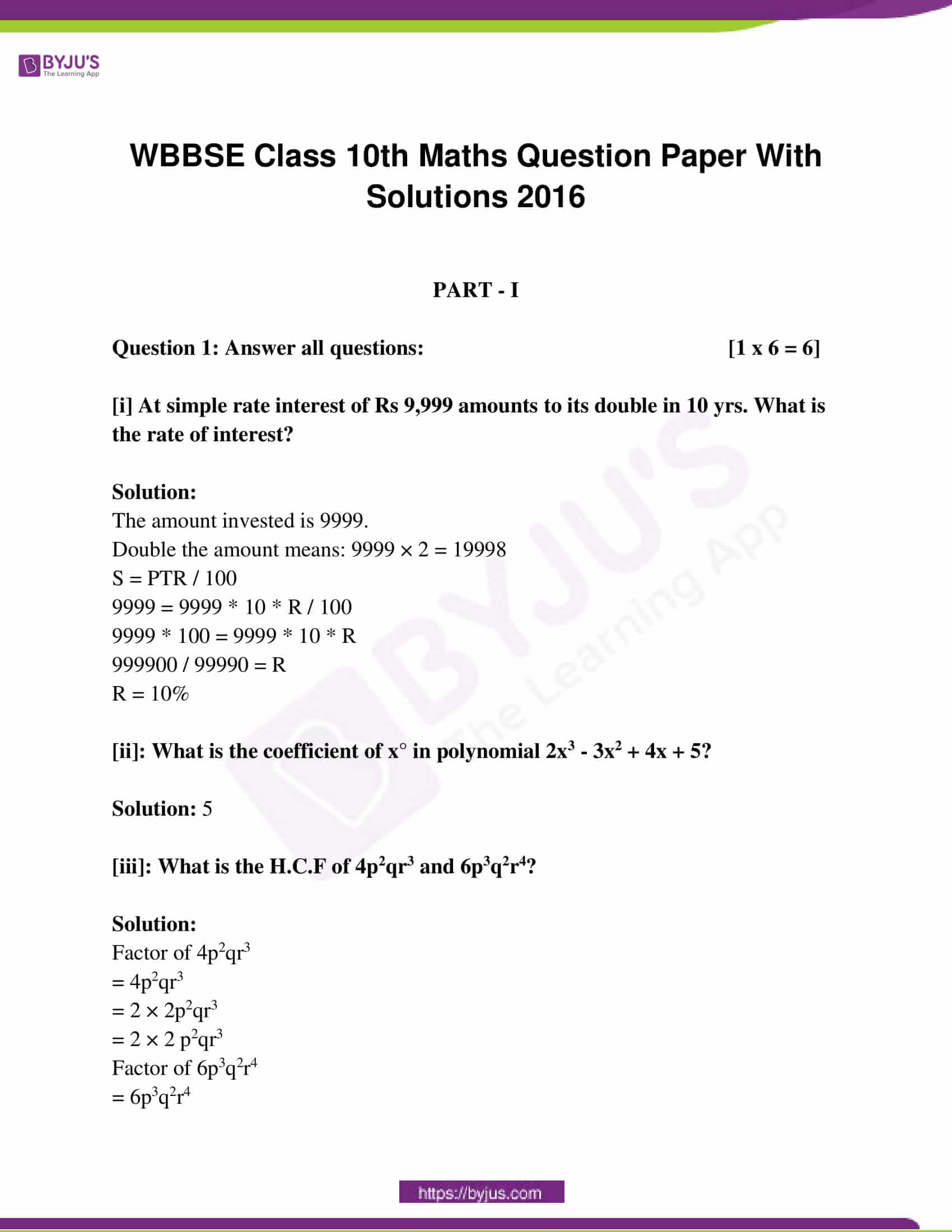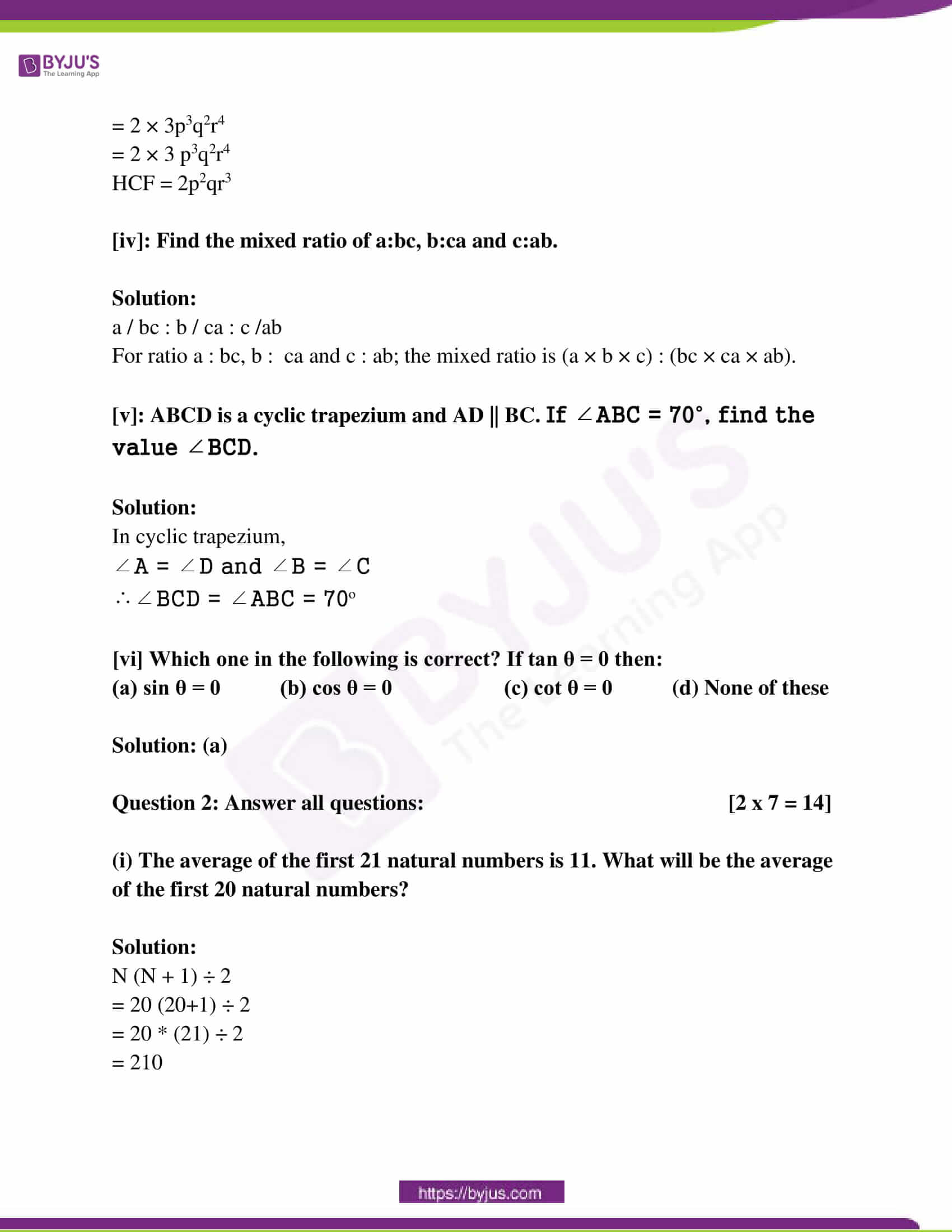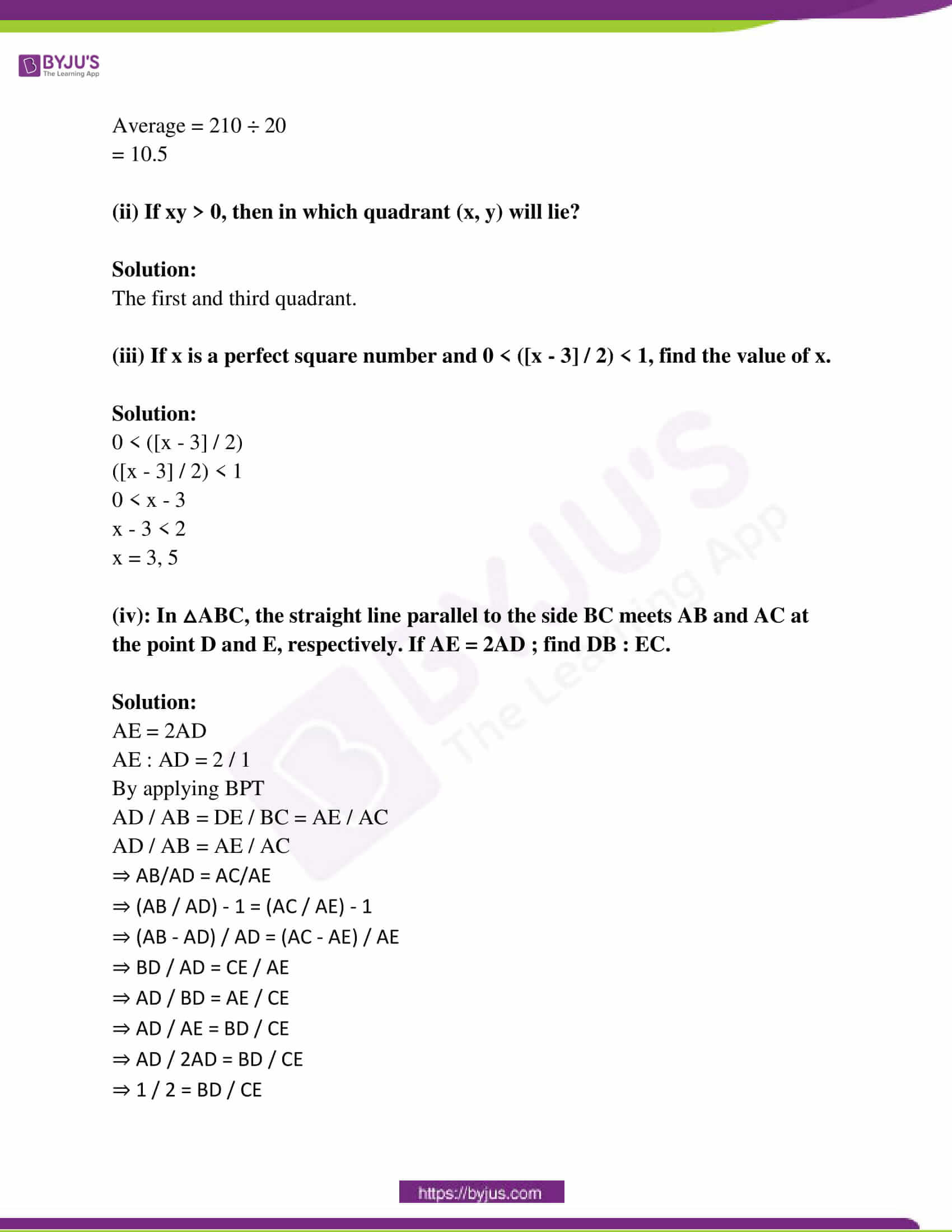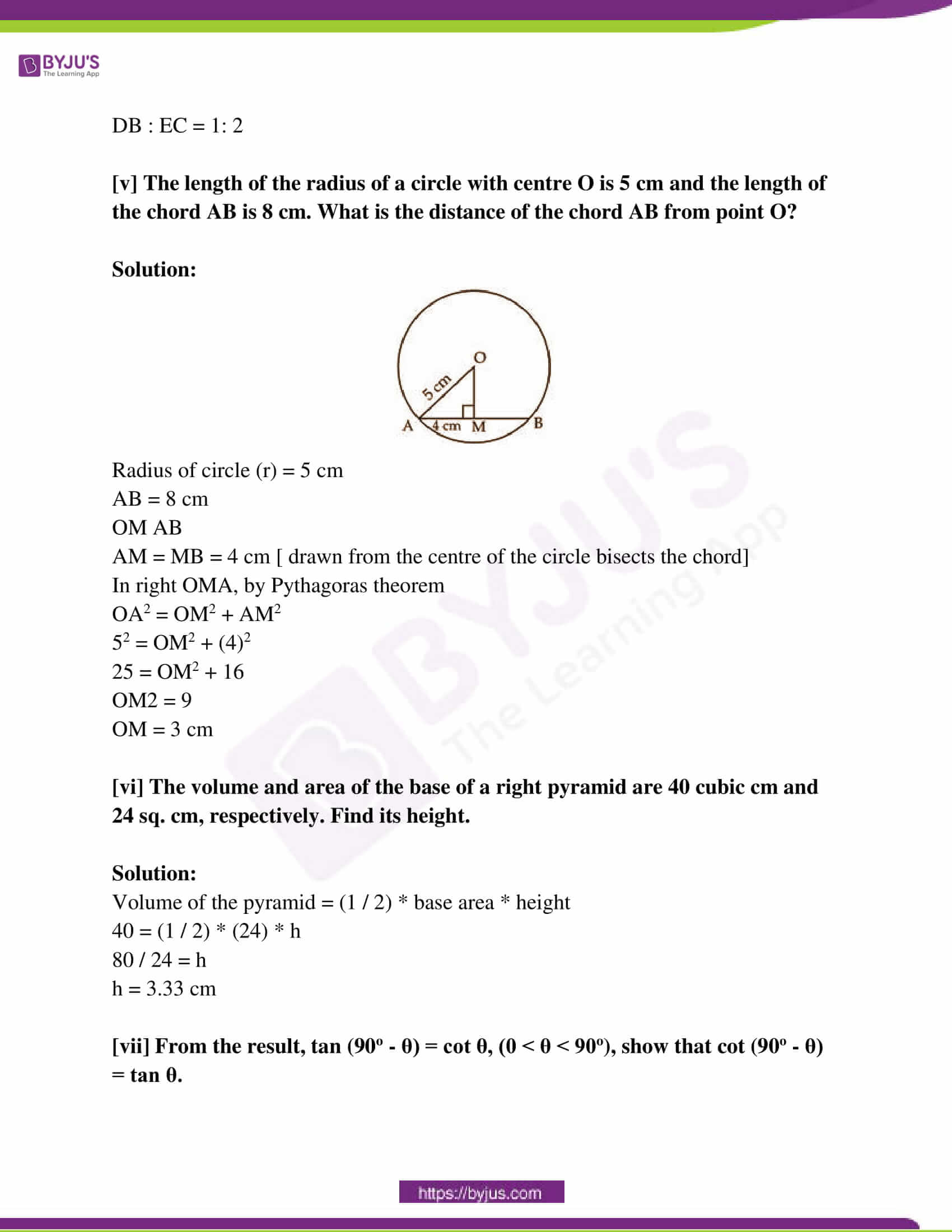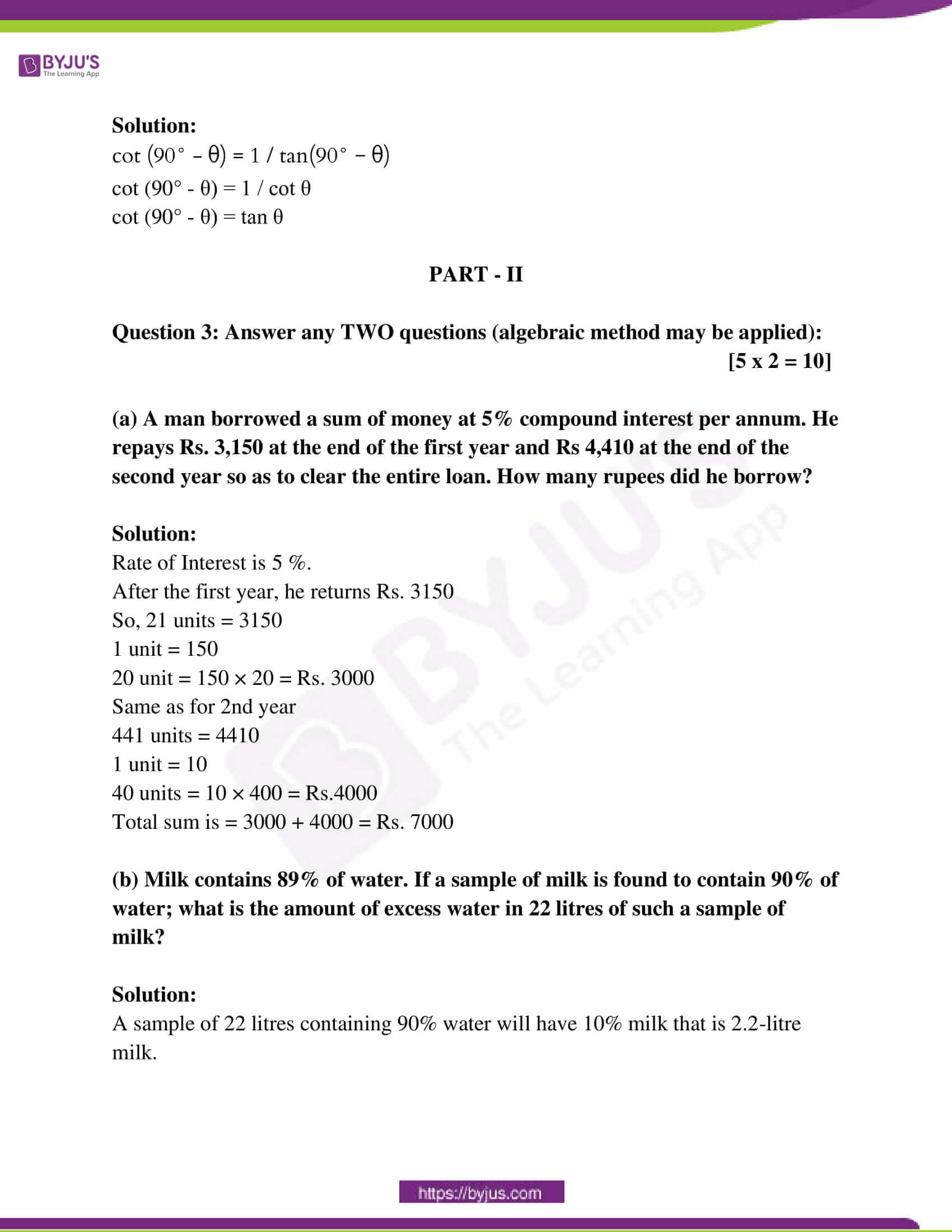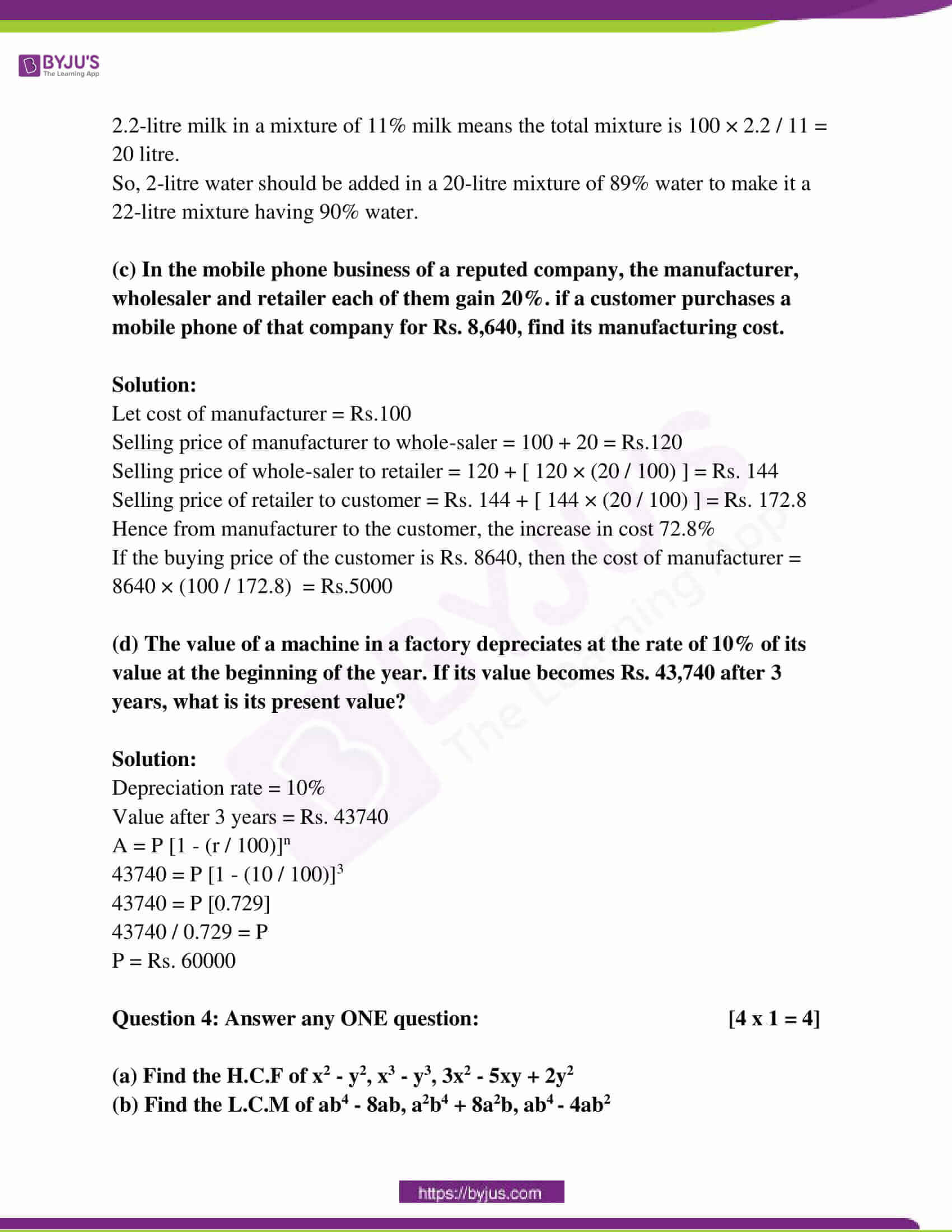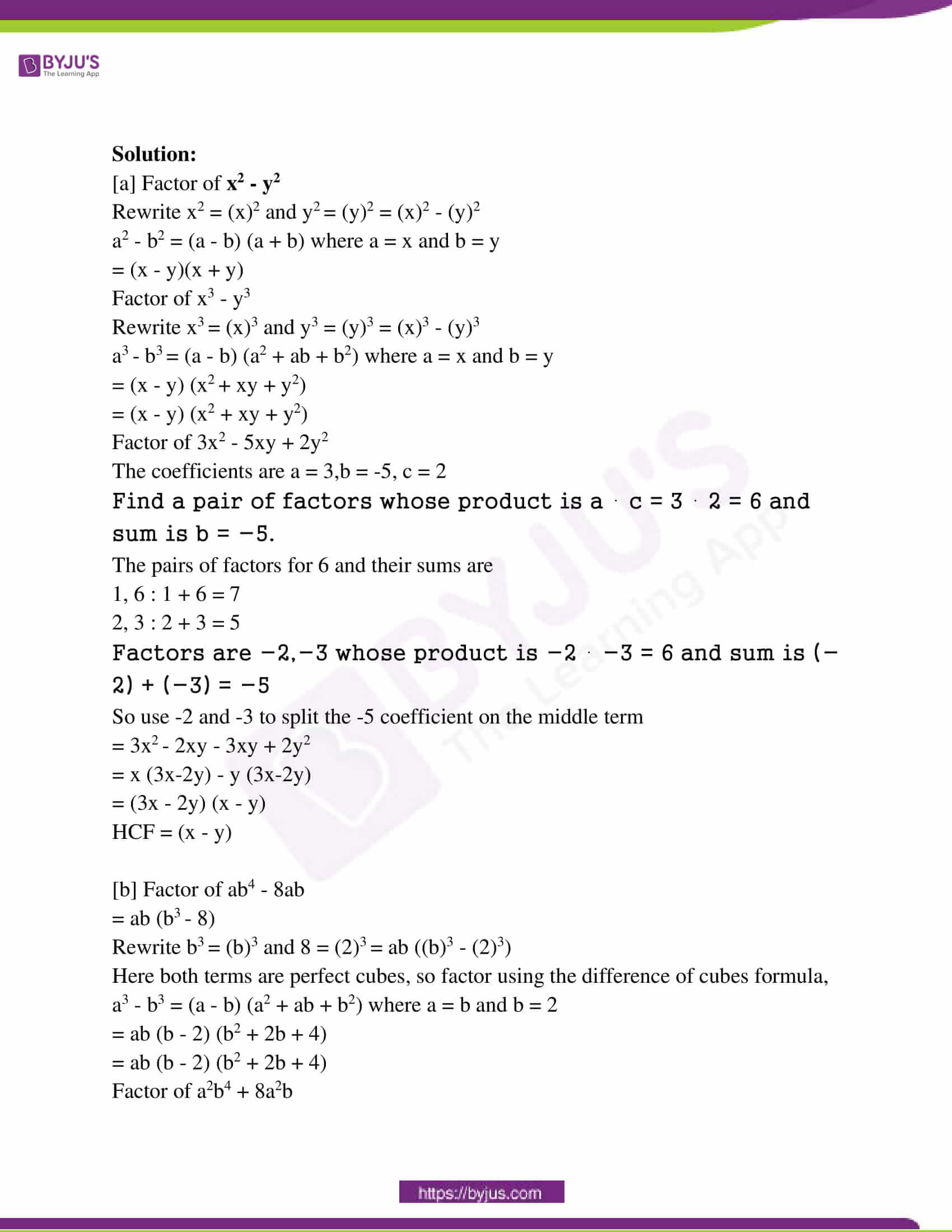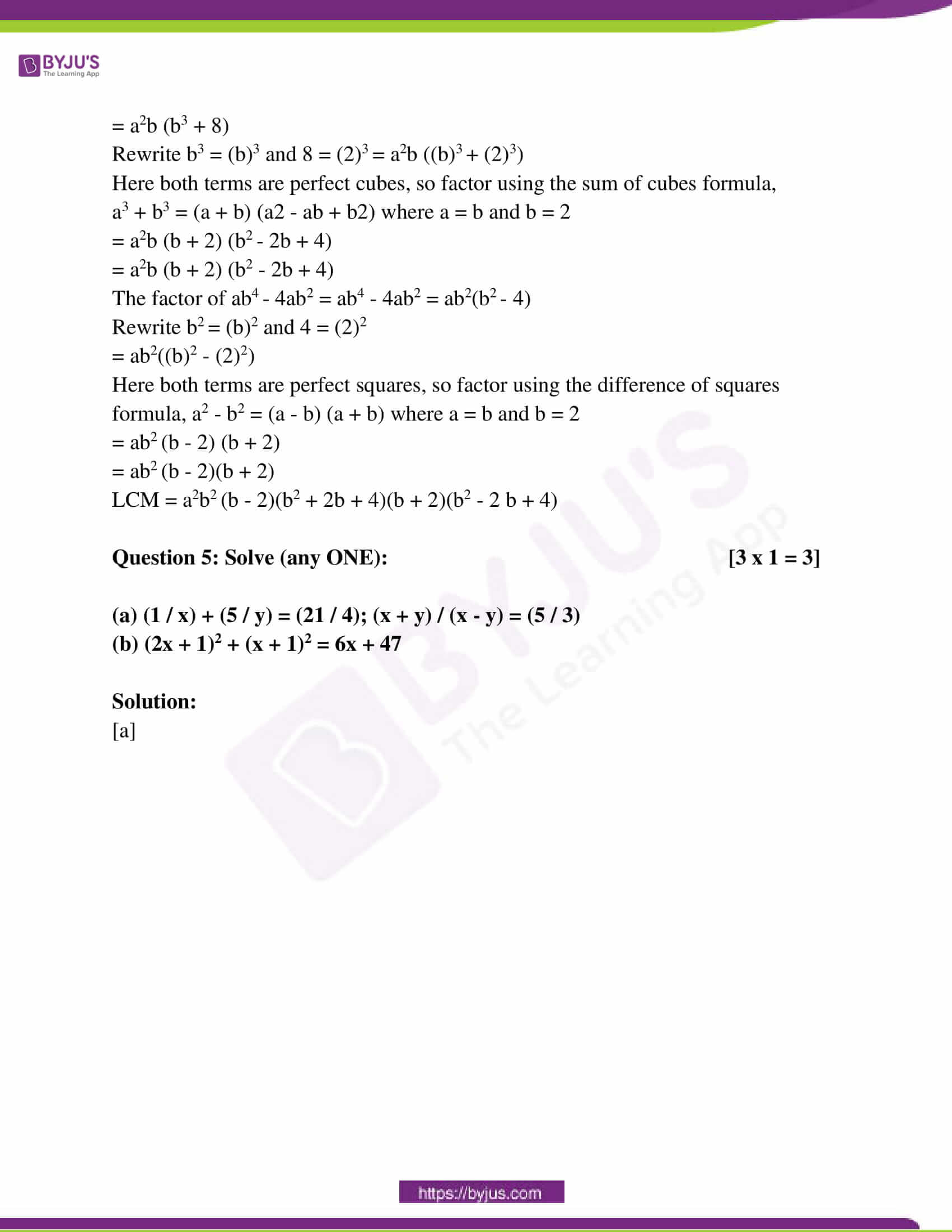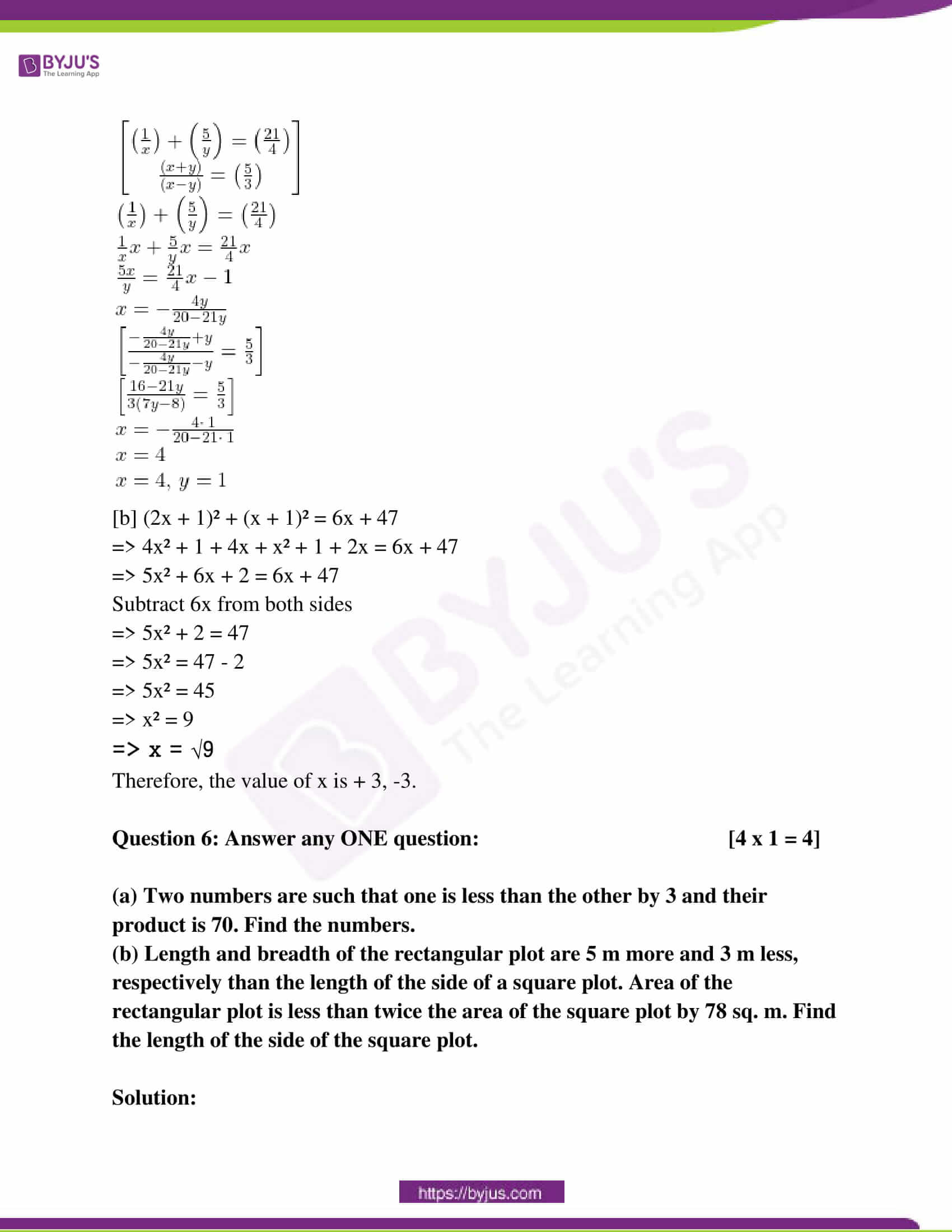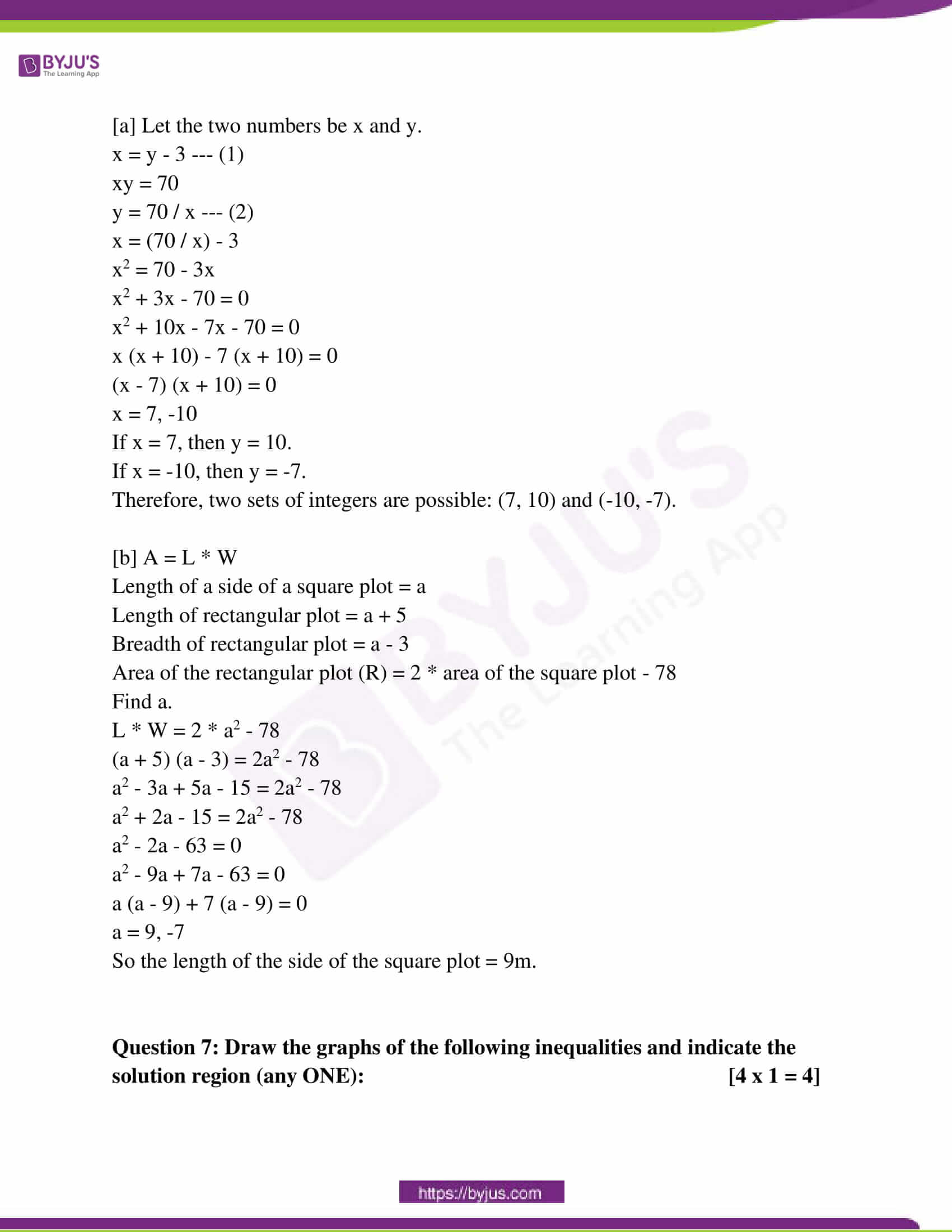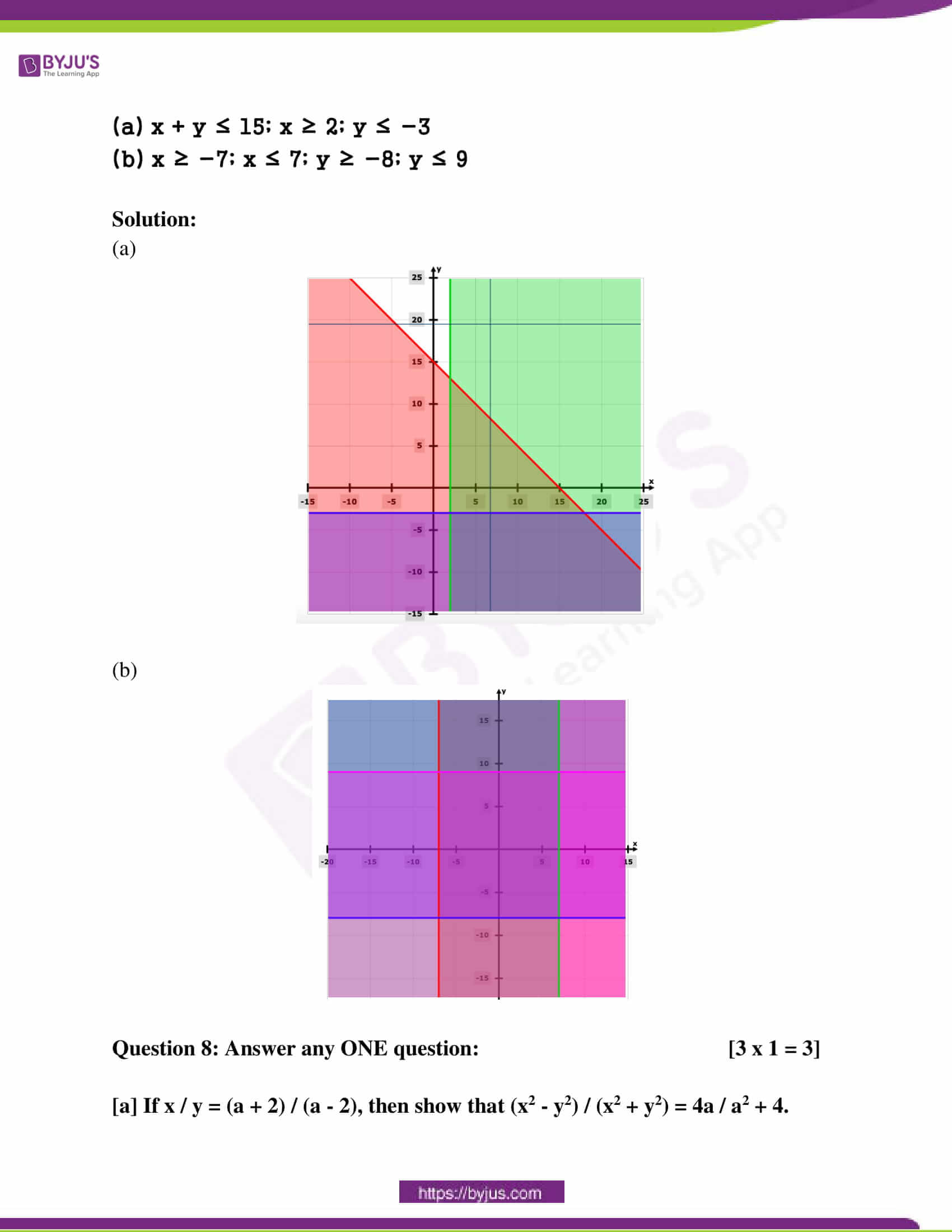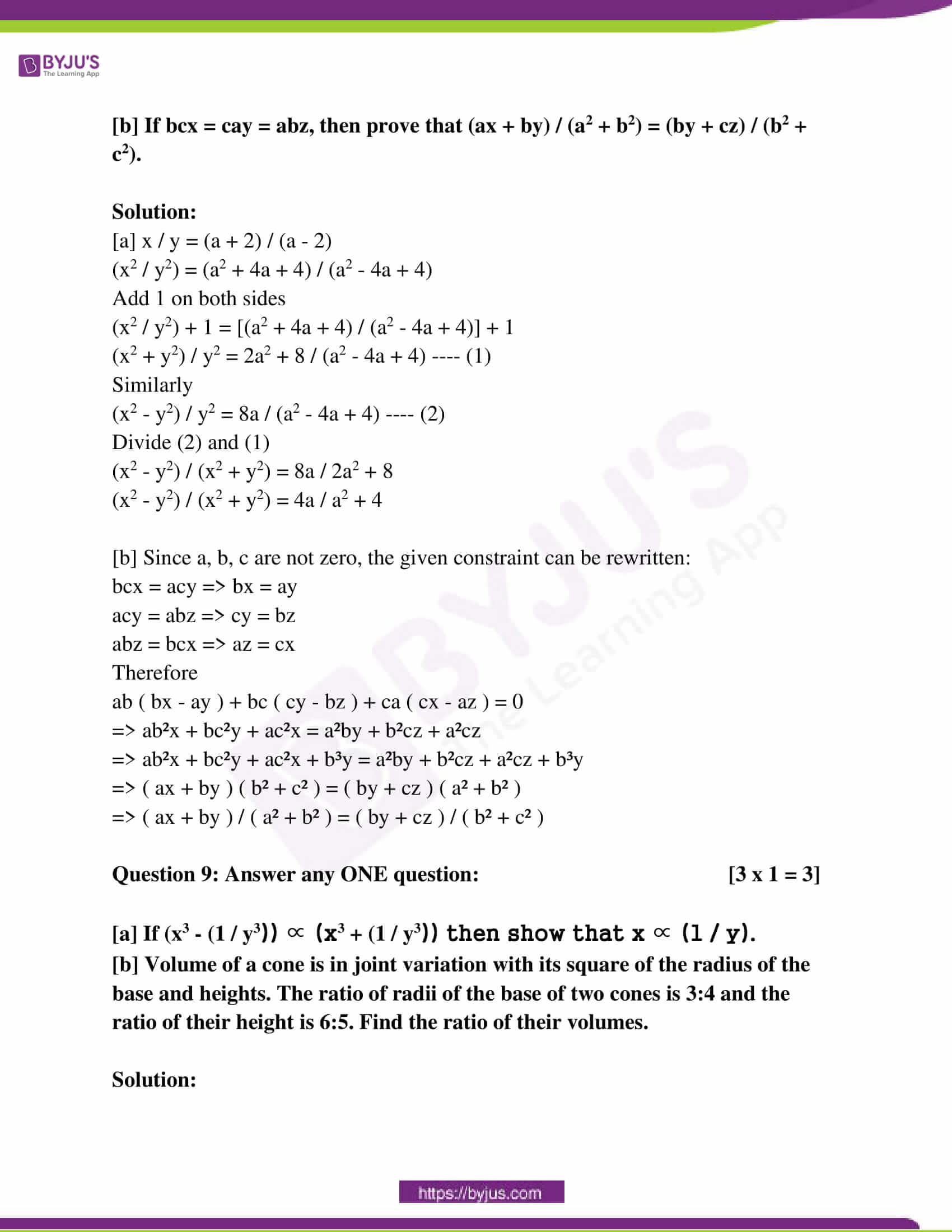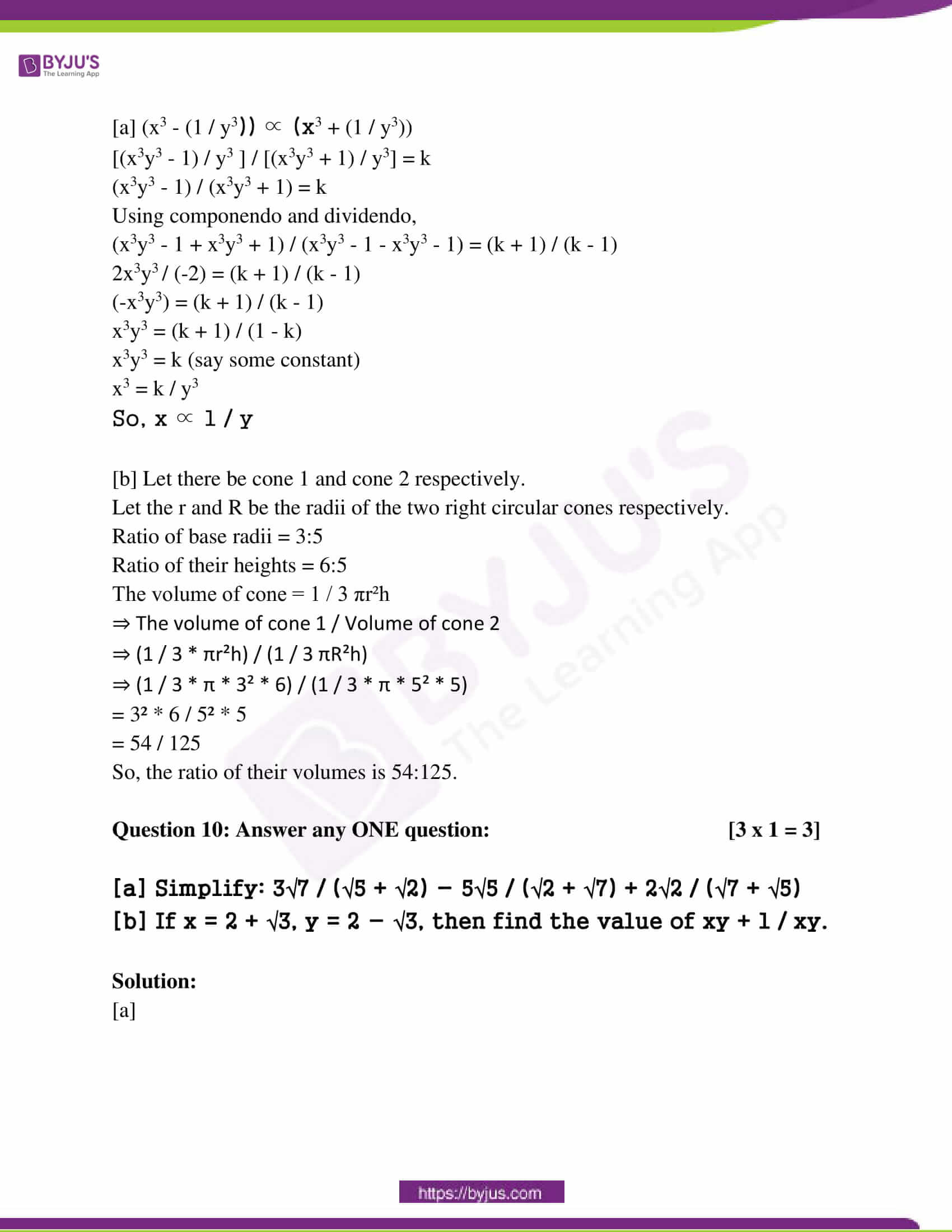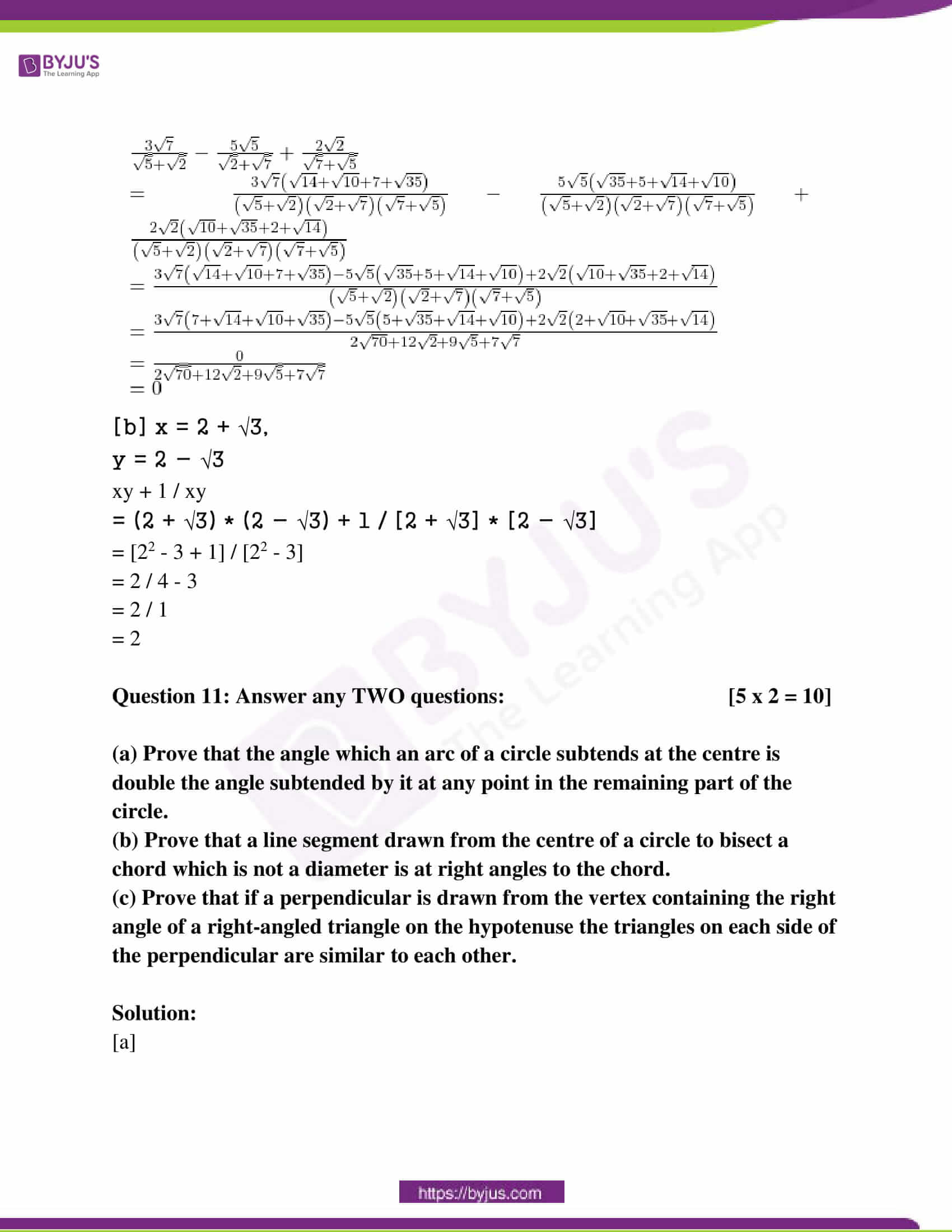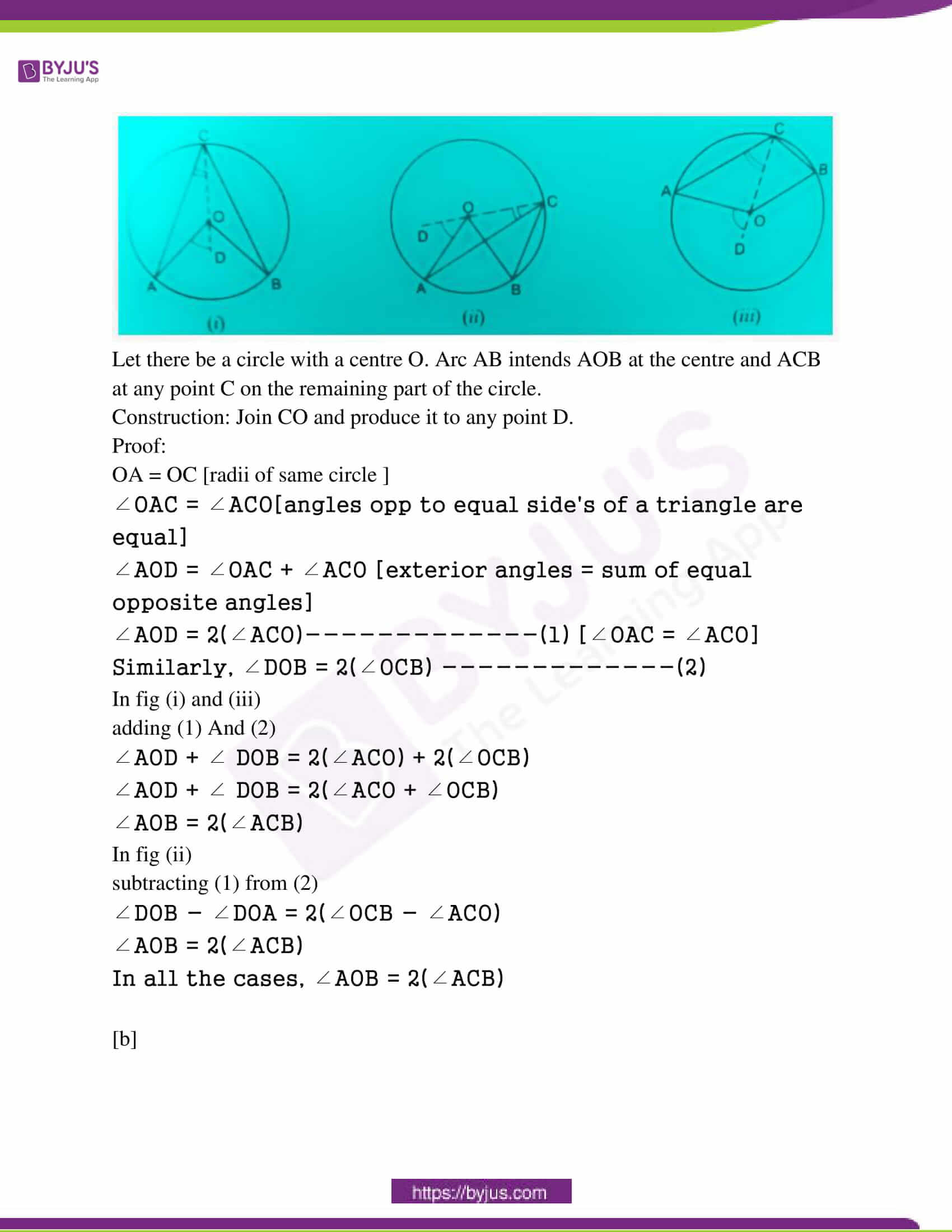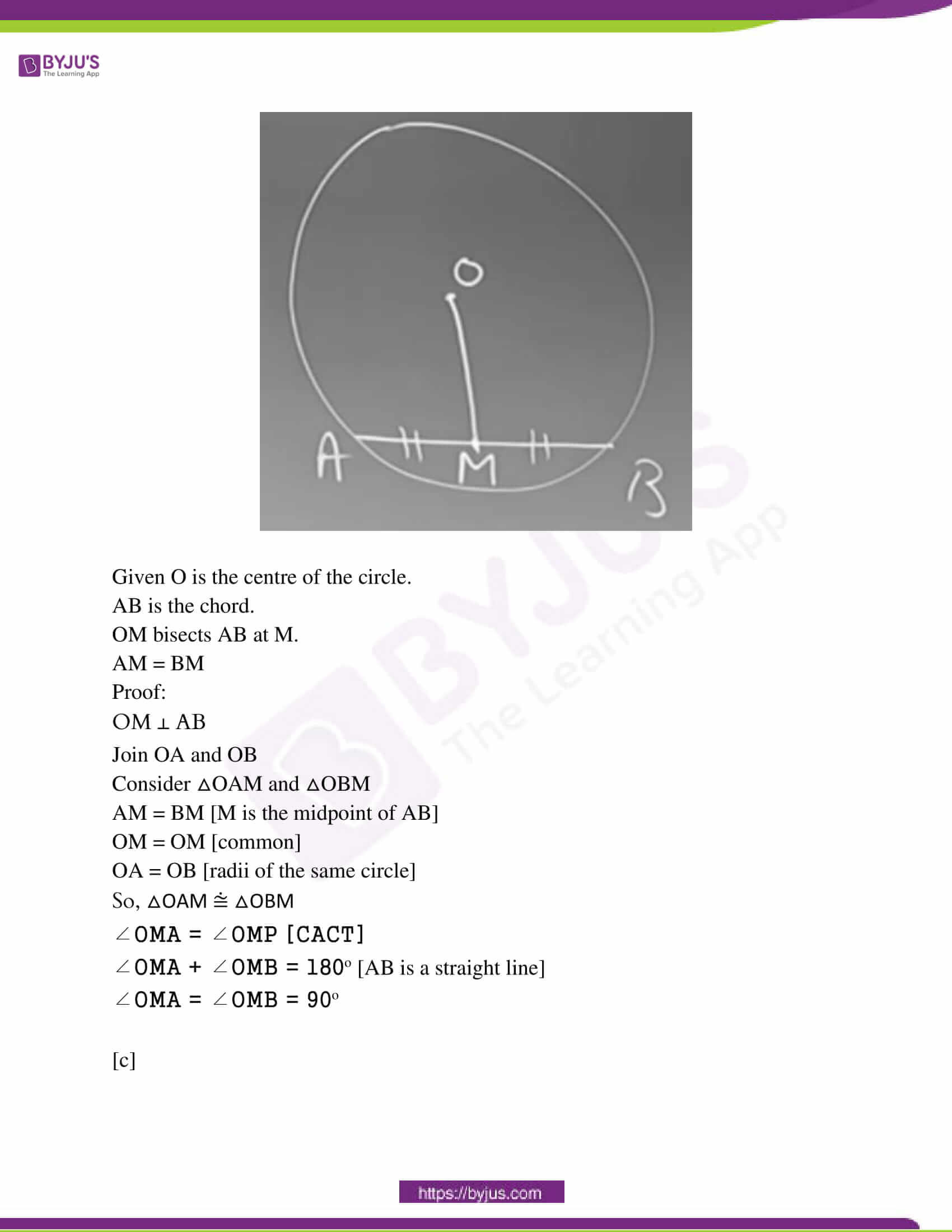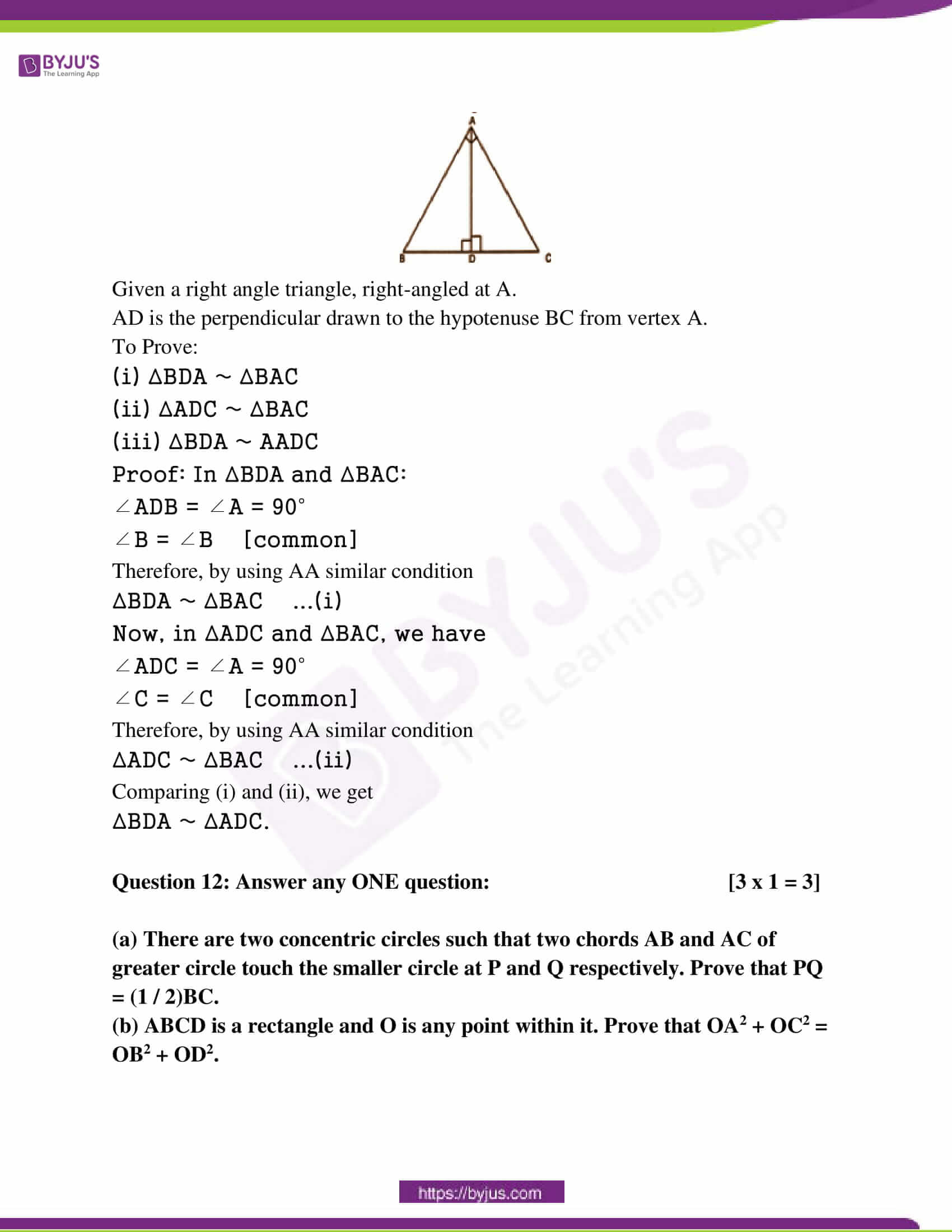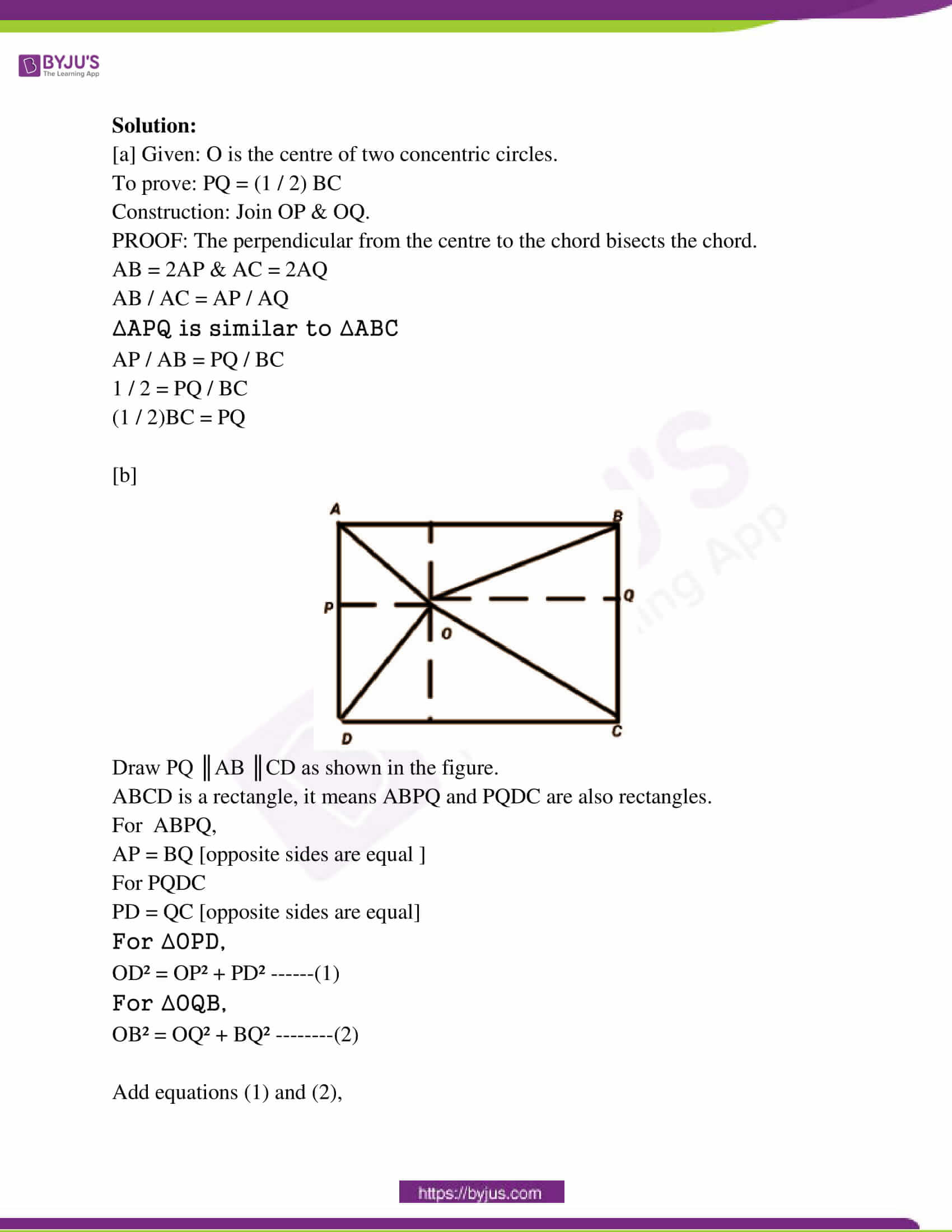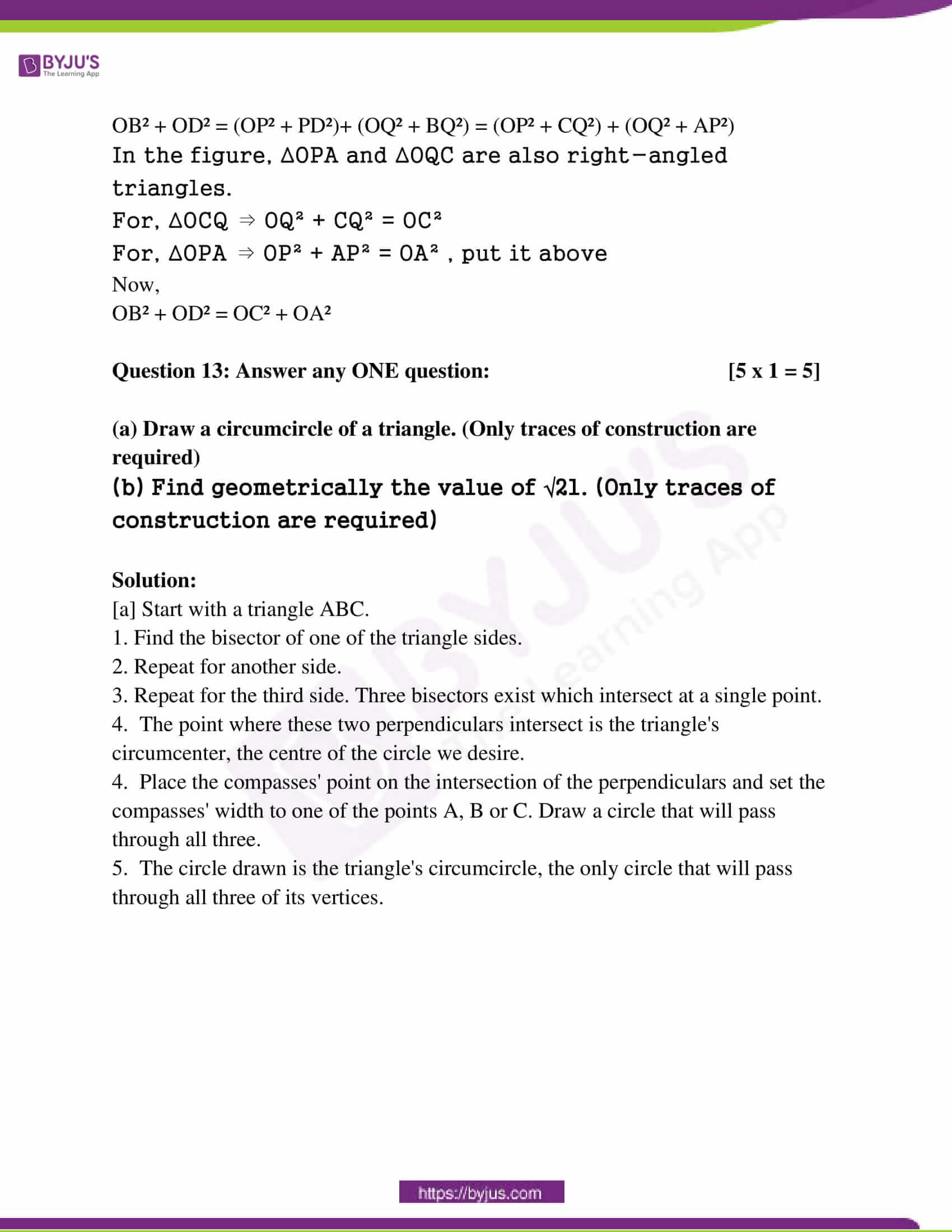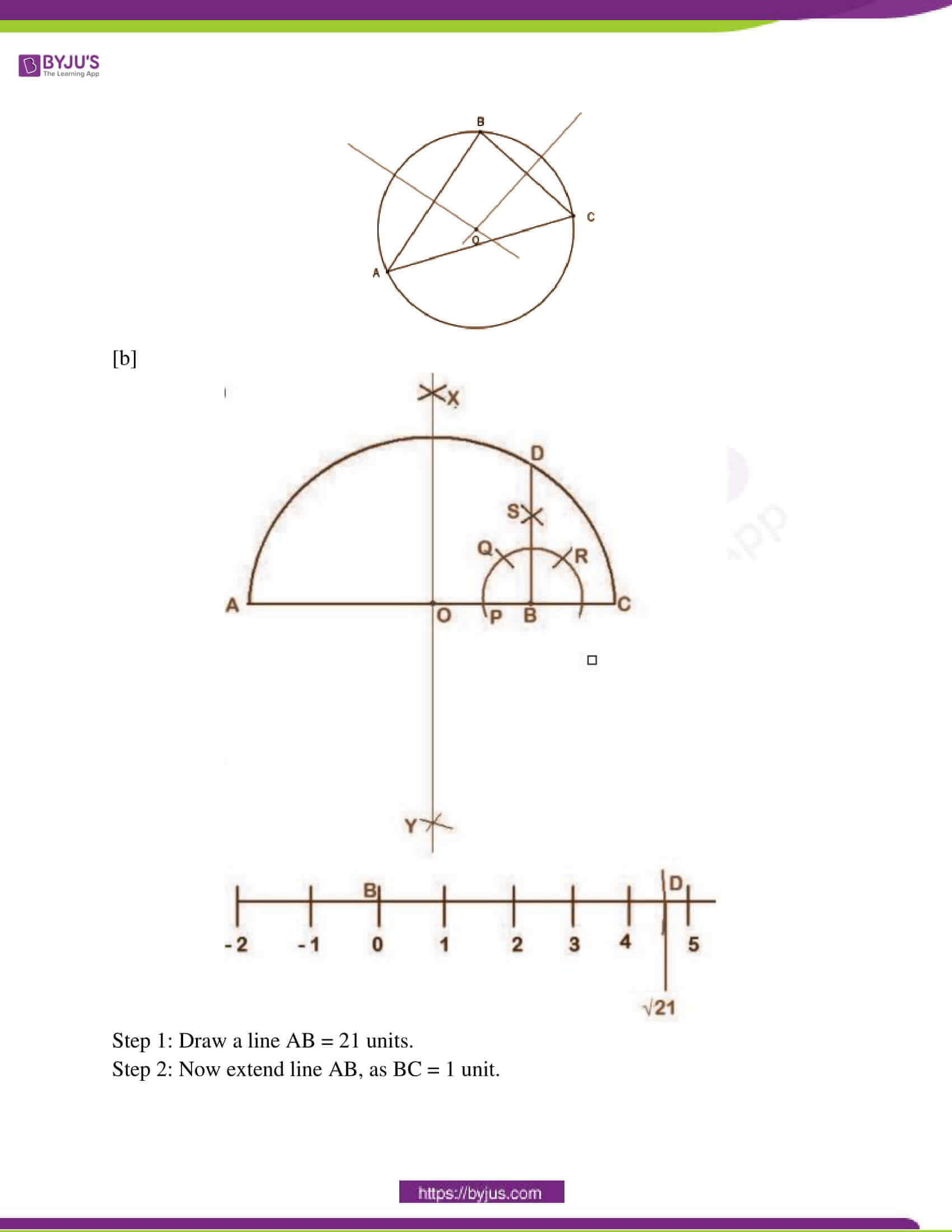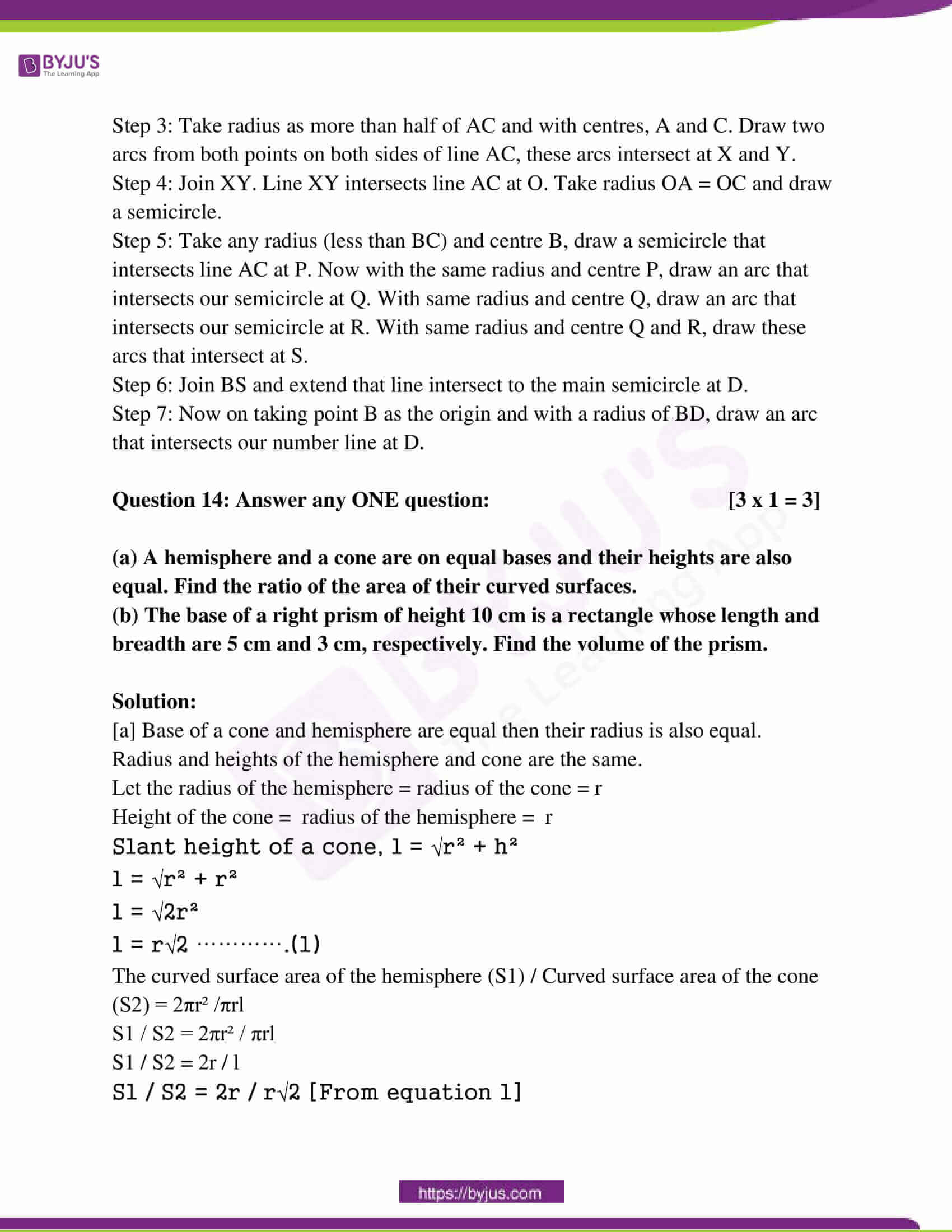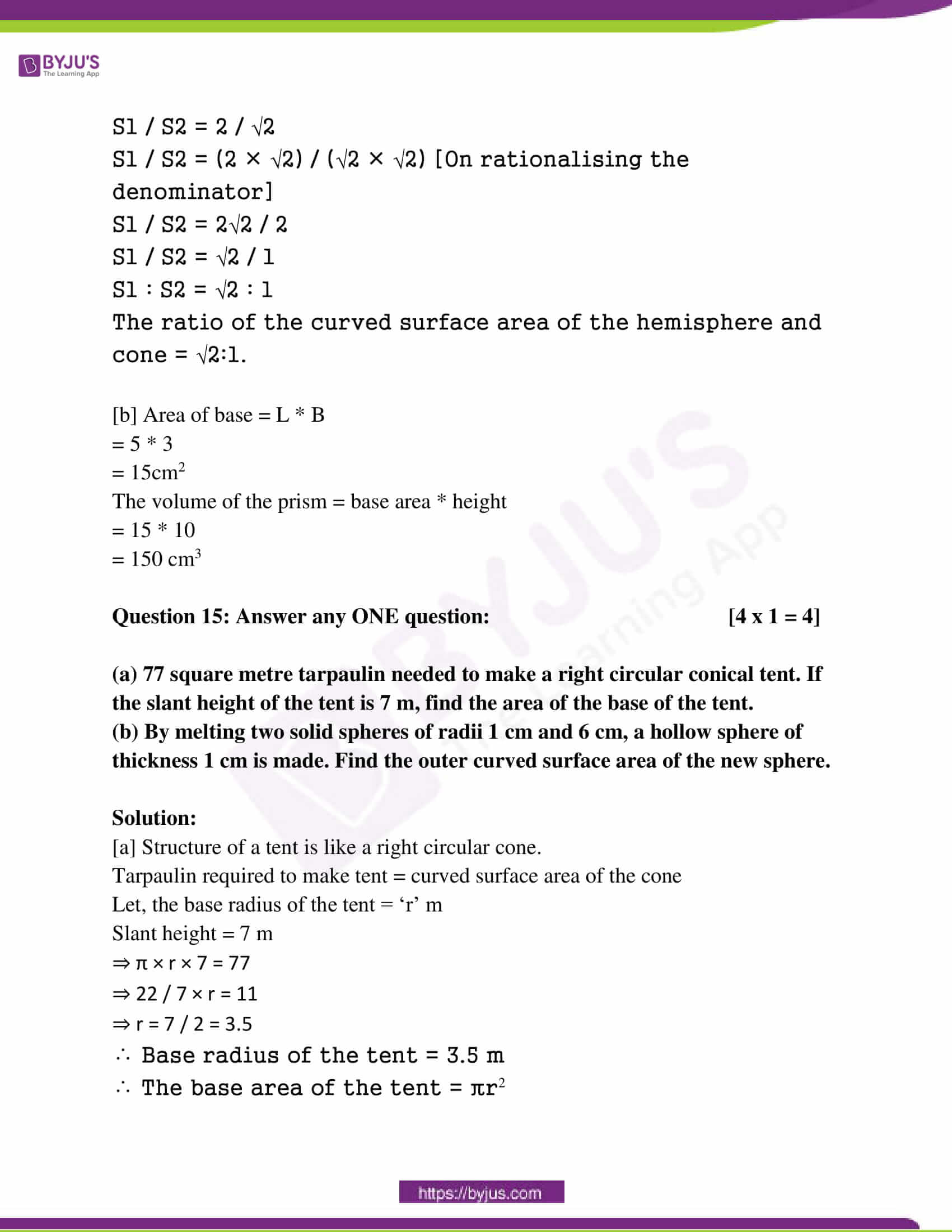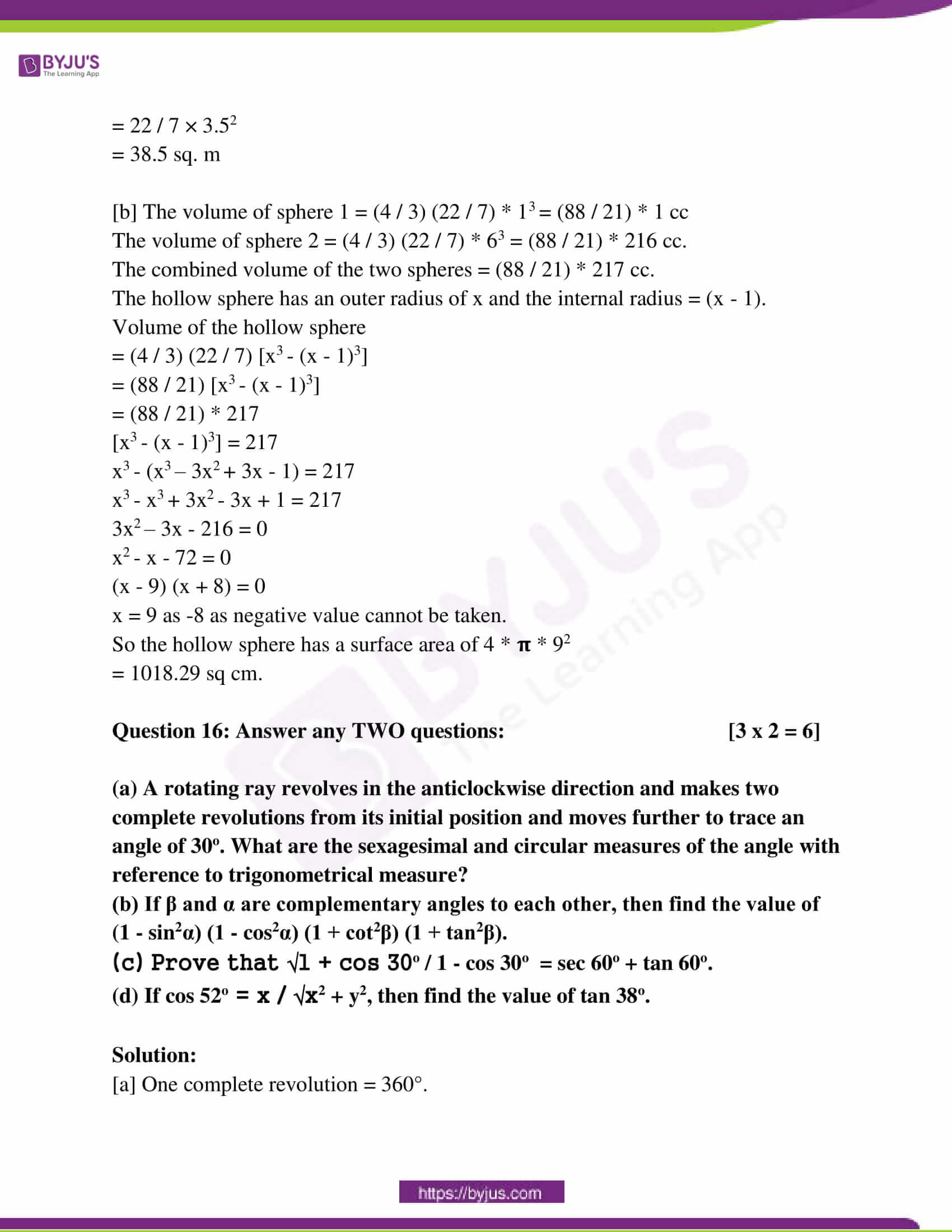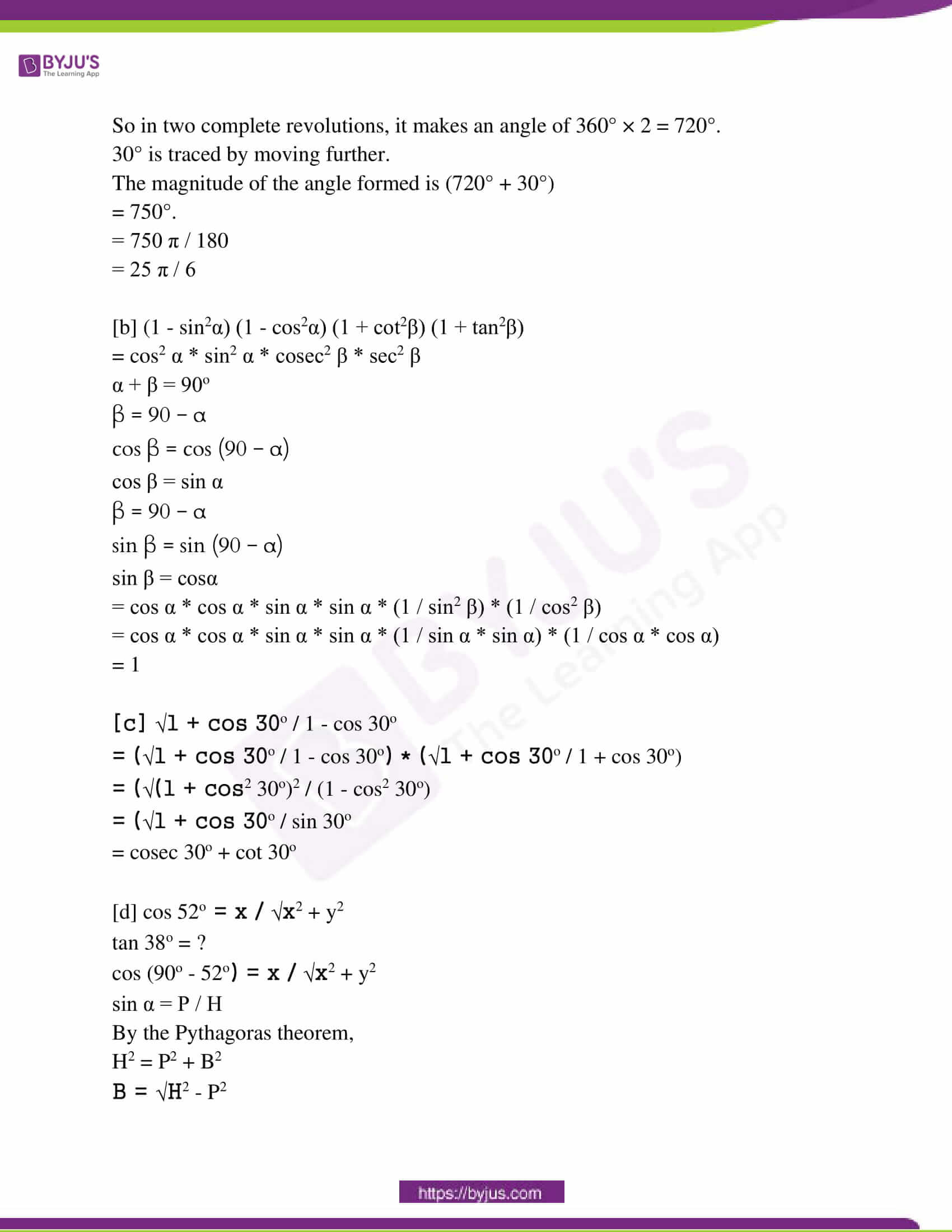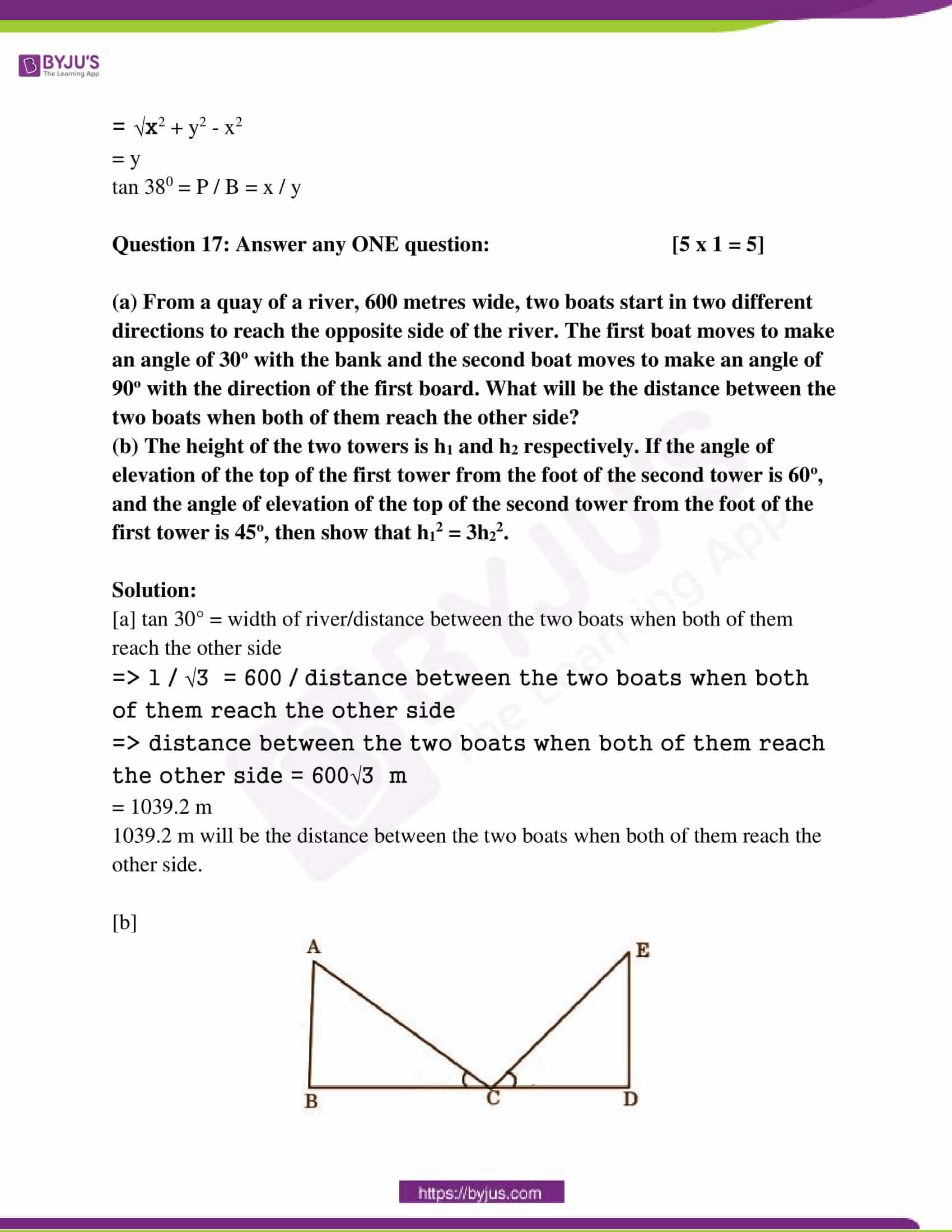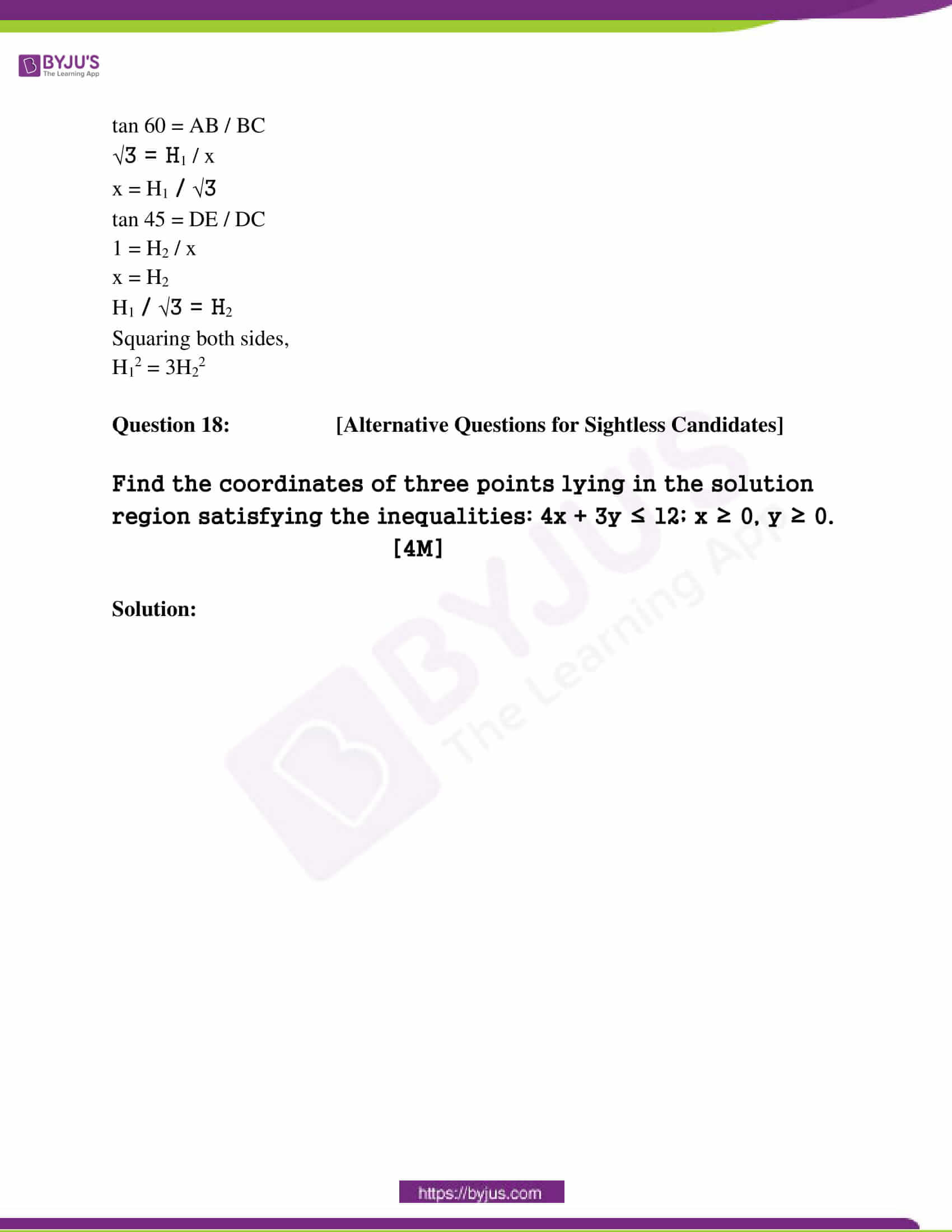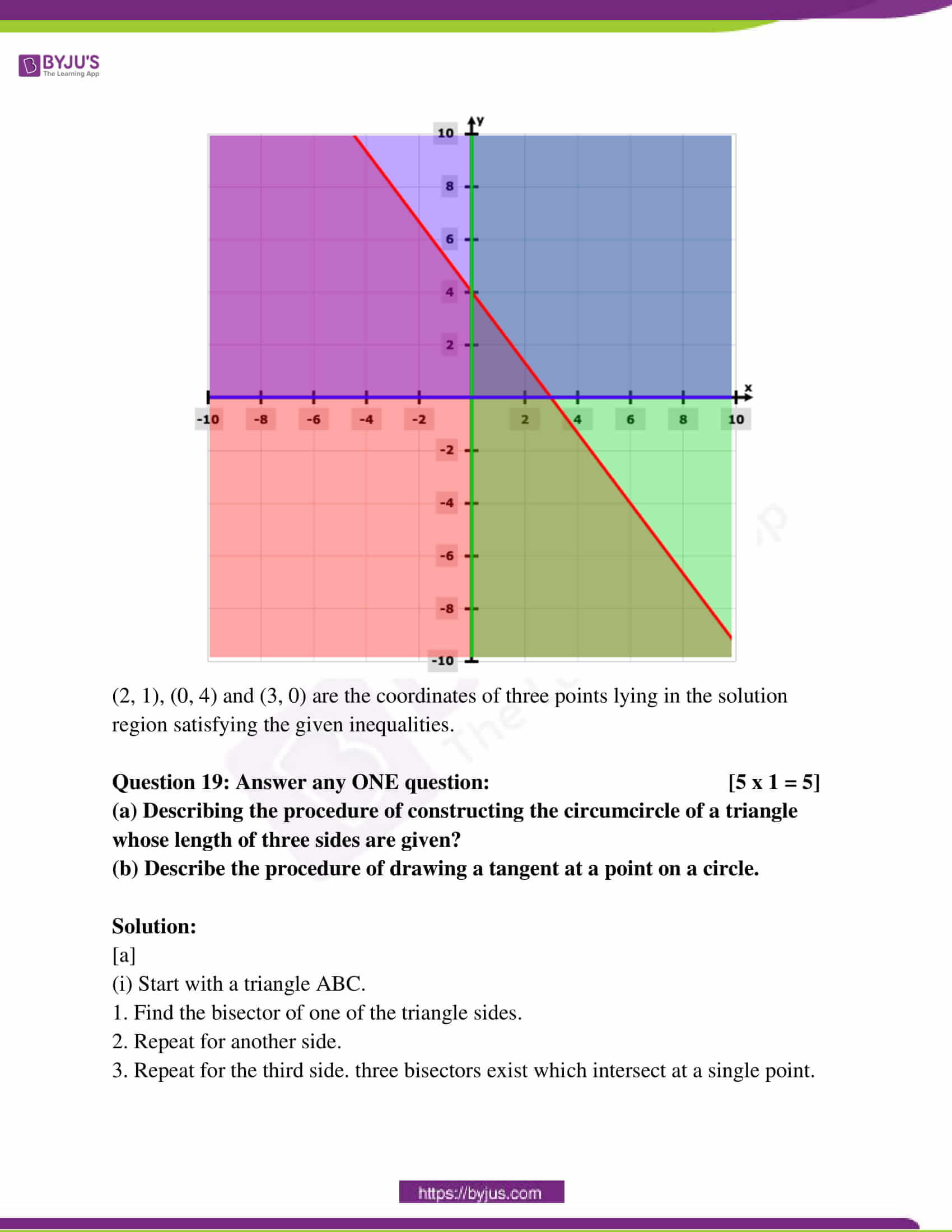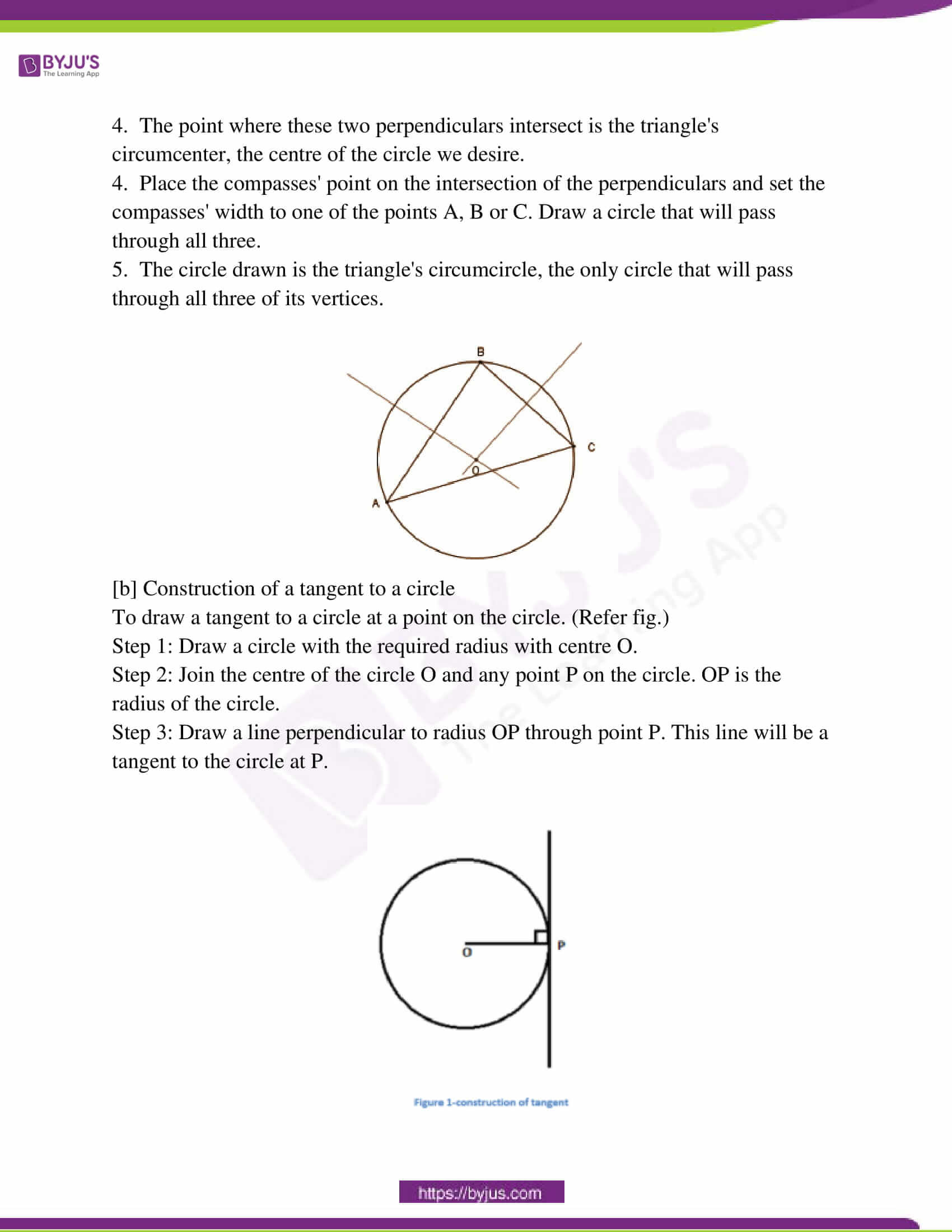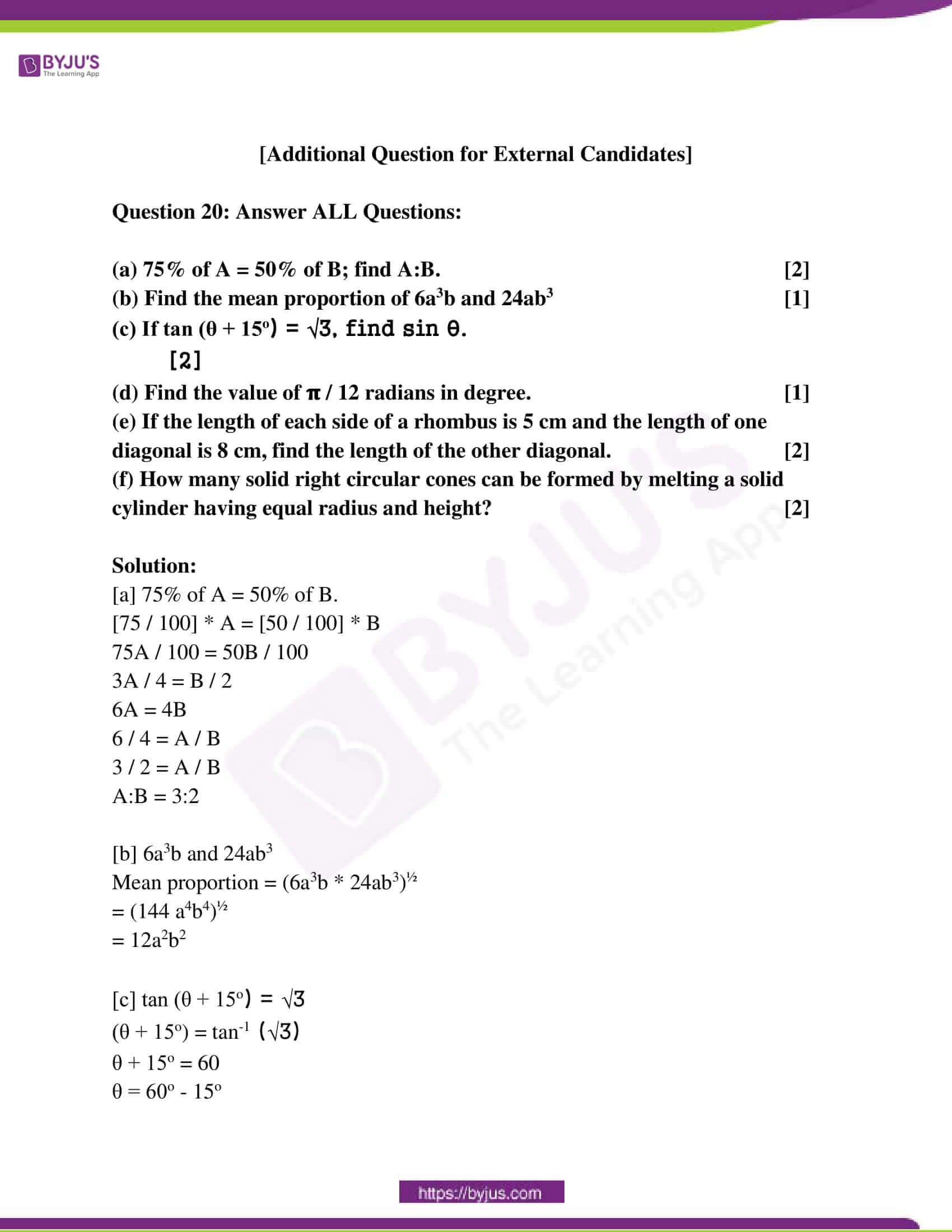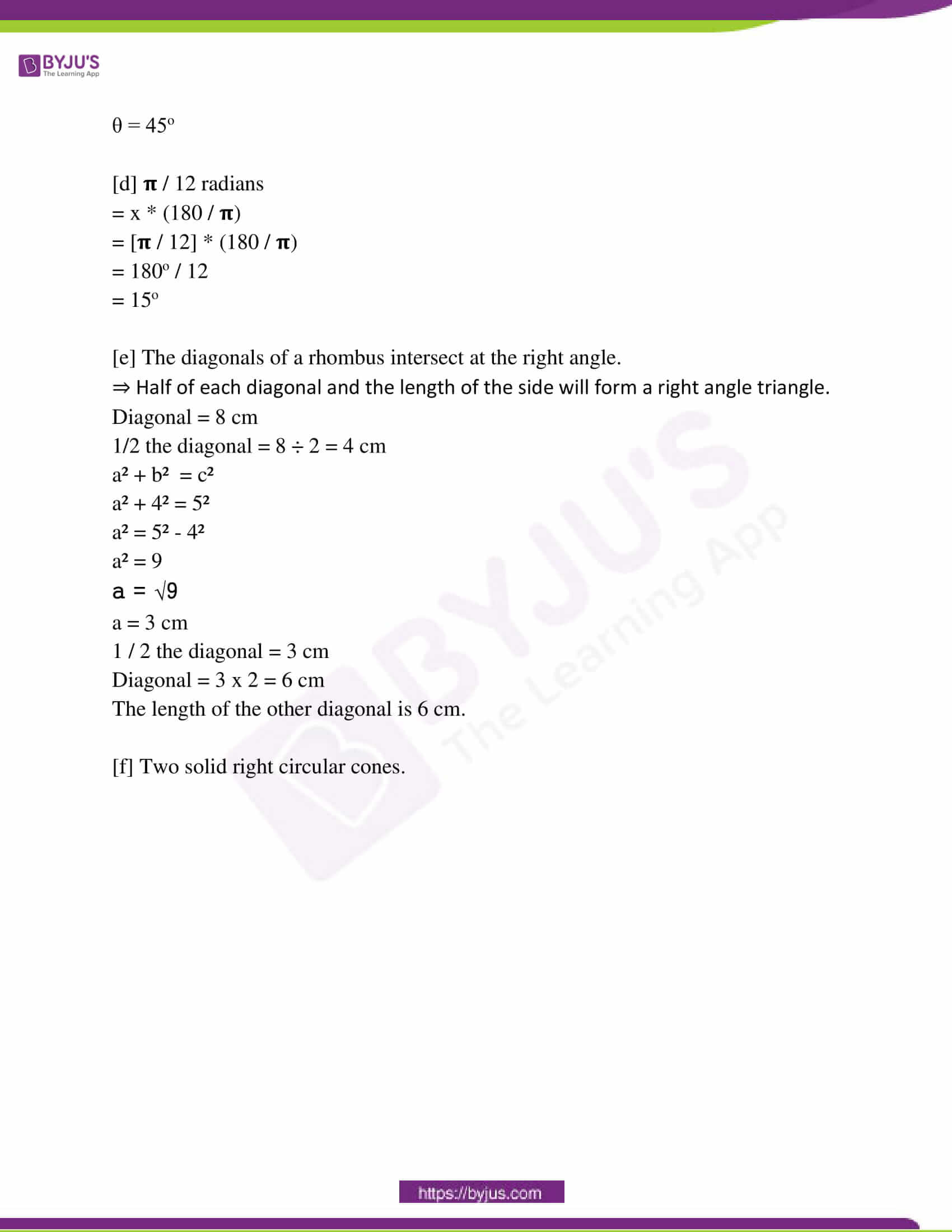PART – I

Question 1: Answer all questions: [1 x 6 = 6]

[i] At simple rate interest of Rs 9,999 amounts to its double in 10 yrs. What is the rate of interest?

Solution:

The amount invested is 9999.

Double the amount means: 9999 × 2 = 19998

S = PTR / 100

9999 = 9999 * 10 * R / 100

9999 * 100 = 9999 * 10 * R

999900 / 99990 = R

R = 10%

[ii]: What is the coefficient of x° in polynomial 2x3 – 3x2 + 4x + 5?

Solution: 5

[iii]: What is the H.C.F of 4p2qr3 and 6p3q2r4?

Solution:

Factor of 4p2qr3

= 4p2qr3

= 2 × 2p2qr3

= 2 × 2 p2qr3

Factor of 6p3q2r4

= 6p3q2r4

= 2 × 3p3q2r4

= 2 × 3 p3q2r4

HCF = 2p2qr3

[iv]: Find the mixed ratio of a:bc, b:ca and c:ab.

Solution:

a / bc : b / ca : c /ab

For ratio a : bc, b : ca and c : ab; the mixed ratio is (a × b × c) : (bc × ca × ab).

[v]: ABCD is a cyclic trapezium and AD || BC. If ∠ABC = 70°, find the value ∠BCD.

Solution:

In cyclic trapezium,

∠A = ∠D and ∠B = ∠C

∴∠BCD = ∠ABC = 70o

[vi] Which one in the following is correct? If tan θ = 0 then:

(a) sin θ = 0 (b) cos θ = 0 (c) cot θ = 0 (d) None of these

Solution: (a)

Question 2: Answer all questions: [2 x 7 = 14]

(i) The average of the first 21 natural numbers is 11. What will be the average of the first 20 natural numbers?

Solution:

N (N + 1) ÷ 2

= 20 (20+1) ÷ 2

= 20 * (21) ÷ 2

= 210

Average = 210 ÷ 20

= 10.5

(ii) If xy > 0, then in which quadrant (x, y) will lie?

Solution:

(iii) If x is a perfect square number and 0 < ([x – 3] / 2) < 1, find the value of x.

Solution:

0 < ([x – 3] / 2)

([x – 3] / 2) < 1

0 < x – 3

x – 3 < 2

x = 3, 5

(iv): In △ABC, the straight line parallel to the side BC meets AB and AC at the point D and E, respectively. If AE = 2AD ; find DB : EC.

Solution:

AE : AD = 2 / 1

By applying BPT

AD / AB = DE / BC = AE / AC

AD / AB = AE / AC

⇒ (AB / AD) – 1 = (AC / AE) – 1

⇒ (AB – AD) / AD = (AC – AE) / AE

⇒ BD / AD = CE / AE

⇒ AD / BD = AE / CE

⇒ AD / AE = BD / CE

⇒ 1 / 2 = BD / CE

DB : EC = 1: 2

[v] The length of the radius of a circle with centre O is 5 cm and the length of the chord AB is 8 cm. What is the distance of the chord AB from point O?

Solution: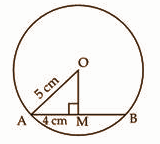Radius of circle (r) = 5 cm

AB = 8 cm

OM AB

AM = MB = 4 cm [ drawn from the centre of the circle bisects the chord]

In right OMA, by Pythagoras theorem

OA2 = OM2 + AM2

52 = OM2 + (4)2

25 = OM2 + 16

OM2 = 9

OM = 3 cm

[vi] The volume and area of the base of a right pyramid are 40 cubic cm and 24 sq. cm, respectively. Find its height.

Solution:

Volume of the pyramid = (1 / 2) * base area * height

40 = (1 / 2) * (24) * h

80 / 24 = h

h = 3.33 cm

[vii] From the result, tan (90o – θ) = cot θ, (0 < θ < 90o), show that cot (90o – θ) = tan θ.

Solution:

cot (90° – θ) = 1 / tan(90° − θ)

cot (90° – θ) = 1 / cot θ

cot (90° – θ) = tan θ

PART – II

Question 3: Answer any TWO questions (algebraic method may be applied):

[5 x 2 = 10]

(a) A man borrowed a sum of money at 5% compound interest per annum. He repays Rs. 3,150 at the end of the first year and Rs 4,410 at the end of the second year so as to clear the entire loan. How many rupees did he borrow?

Solution:

Rate of Interest is 5 %.

After the first year, he returns Rs. 3150

So, 21 units = 3150

1 unit = 150

20 unit = 150 × 20 = Rs. 3000

Same as for 2nd year

441 units = 4410

1 unit = 10

40 units = 10 × 400 = Rs.4000

Total sum is = 3000 + 4000 = Rs. 7000

(b) Milk contains 89% of water. If a sample of milk is found to contain 90% of water; what is the amount of excess water in 22 litres of such a sample of milk?

Solution:

A sample of 22 litres containing 90% water will have 10% milk that is 2.2-litre milk.

2.2-litre milk in a mixture of 11% milk means the total mixture is 100 × 2.2 / 11 = 20 litre.

So, 2-litre water should be added in a 20-litre mixture of 89% water to make it a 22-litre mixture having 90% water.

(c) In the mobile phone business of a reputed company, the manufacturer, wholesaler and retailer each of them gain 20%. if a customer purchases a mobile phone of that company for Rs. 8,640, find its manufacturing cost.

Solution:

Let cost of manufacturer = Rs.100

Selling price of manufacturer to whole-saler = 100 + 20 = Rs.120

Selling price of whole-saler to retailer = 120 + [ 120 × (20 / 100) ] = Rs. 144

Selling price of retailer to customer = Rs. 144 + [ 144 × (20 / 100) ] = Rs. 172.8

Hence from manufacturer to the customer, the increase in cost 72.8%

If the buying price of the customer is Rs. 8640, then the cost of manufacturer = 8640 × (100 / 172.8) = Rs.5000

(d) The value of a machine in a factory depreciates at the rate of 10% of its value at the beginning of the year. If its value becomes Rs. 43,740 after 3 years, what is its present value?

Solution:

Depreciation rate = 10%

Value after 3 years = Rs. 43740

A = P [1 – (r / 100)]n

43740 = P [1 – (10 / 100)]3

43740 = P [0.729]

43740 / 0.729 = P

P = Rs. 60000

Question 4: Answer any ONE question: [4 x 1 = 4]

(a) Find the H.C.F of x2 – y2, x3 – y3, 3x2 – 5xy + 2y2

(b) Find the L.C.M of ab4 – 8ab, a2b4 + 8a2b, ab4 – 4ab2

Solution:

[a] Factor of x2 – y2

Rewrite x2 = (x)2 and y2 = (y)2 = (x)2 – (y)2

a2 – b2 = (a – b) (a + b) where a = x and b = y

= (x – y)(x + y)

Factor of x3 – y3

Rewrite x3 = (x)3 and y3 = (y)3 = (x)3 – (y)3

a3 – b3 = (a – b) (a2 + ab + b2) where a = x and b = y

= (x – y) (x2 + xy + y2)

= (x – y) (x2 + xy + y2)

Factor of 3x2 – 5xy + 2y2

The coefficients are a = 3,b = -5, c = 2

Find a pair of factors whose product is a ⋅ c = 3 ⋅ 2 = 6 and sum is b = -5.

The pairs of factors for 6 and their sums are

1, 6 : 1 + 6 = 7

2, 3 : 2 + 3 = 5

Factors are -2,-3 whose product is -2 ⋅ -3 = 6 and sum is (-2) + (-3) = -5

So use -2 and -3 to split the -5 coefficient on the middle term

= 3x2 – 2xy – 3xy + 2y2

= x (3x-2y) – y (3x-2y)

= (3x – 2y) (x – y)

HCF = (x – y)

[b] Factor of ab4 – 8ab

= ab (b3 – 8)

Rewrite b3 = (b)3 and 8 = (2)3 = ab ((b)3 – (2)3)

Here both terms are perfect cubes, so factor using the difference of cubes formula, a3 – b3 = (a – b) (a2 + ab + b2) where a = b and b = 2

= ab (b – 2) (b2 + 2b + 4)

= ab (b – 2) (b2 + 2b + 4)

Factor of a2b4 + 8a2b

= a2b (b3 + 8)

Rewrite b3 = (b)3 and 8 = (2)3 = a2b ((b)3 + (2)3)

Here both terms are perfect cubes, so factor using the sum of cubes formula,

a3 + b3 = (a + b) (a2 – ab + b2) where a = b and b = 2

= a2b (b + 2) (b2 – 2b + 4)

= a2b (b + 2) (b2 – 2b + 4)

The factor of ab4 – 4ab2 = ab4 – 4ab2 = ab2(b2 – 4)

Rewrite b2 = (b)2 and 4 = (2)2

= ab2((b)2 – (2)2)

Here both terms are perfect squares, so factor using the difference of squares formula, a2 – b2 = (a – b) (a + b) where a = b and b = 2

= ab2 (b – 2) (b + 2)

= ab2 (b – 2)(b + 2)

LCM = a2b2 (b – 2)(b2 + 2b + 4)(b + 2)(b2 – 2 b + 4)

Question 5: Solve (any ONE): [3 x 1 = 3]

(a) (1 / x) + (5 / y) = (21 / 4); (x + y) / (x – y) = (5 / 3)

(b) (2x + 1)2 + (x + 1)2 = 6x + 47

Solution:

[a]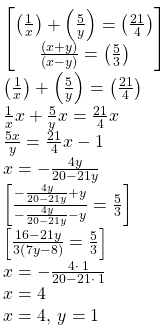[b] (2x + 1)² + (x + 1)² = 6x + 47

=> 4x² + 1 + 4x + x² + 1 + 2x = 6x + 47

=> 5x² + 6x + 2 = 6x + 47

Subtract 6x from both sides

=> 5x² + 2 = 47

=> 5x² = 47 – 2

=> 5x² = 45

=> x² = 9

=> x = √9

Therefore, the value of x is + 3, -3.

Question 6: Answer any ONE question: [4 x 1 = 4]

(a) Two numbers are such that one is less than the other by 3 and their product is 70. Find the numbers.

(b) Length and breadth of the rectangular plot are 5 m more and 3 m less, respectively than the length of the side of a square plot. Area of the rectangular plot is less than twice the area of the square plot by 78 sq. m. Find the length of the side of the square plot.

Solution:

[a] Let the two numbers be x and y.

x = y – 3 — (1)

xy = 70

y = 70 / x — (2)

x = (70 / x) – 3

x2 = 70 – 3x

x2 + 3x – 70 = 0

x2 + 10x – 7x – 70 = 0

x (x + 10) – 7 (x + 10) = 0

(x – 7) (x + 10) = 0

x = 7, -10

If x = 7, then y = 10.

If x = -10, then y = -7.

Therefore, two sets of integers are possible: (7, 10) and (-10, -7).

[b] A = L * W

Length of a side of a square plot = a

Length of rectangular plot = a + 5

Breadth of rectangular plot = a – 3

Area of the rectangular plot (R) = 2 * area of the square plot – 78

Find a.

L * W = 2 * a2 – 78

(a + 5) (a – 3) = 2a2 – 78

a2 – 3a + 5a – 15 = 2a2 – 78

a2 + 2a – 15 = 2a2 – 78

a2 – 2a – 63 = 0

a2 – 9a + 7a – 63 = 0

a (a – 9) + 7 (a – 9) = 0

a = 9, -7

So the length of the side of the square plot = 9m.

Question 7: Draw the graphs of the following inequalities and indicate the solution region (any ONE): [4 x 1 = 4]

(a) x + y ≤ 15; x ≥ 2; y ≤ -3

(b) x ≥ -7; x ≤ 7; y ≥ -8; y ≤ 9

Solution:

(a)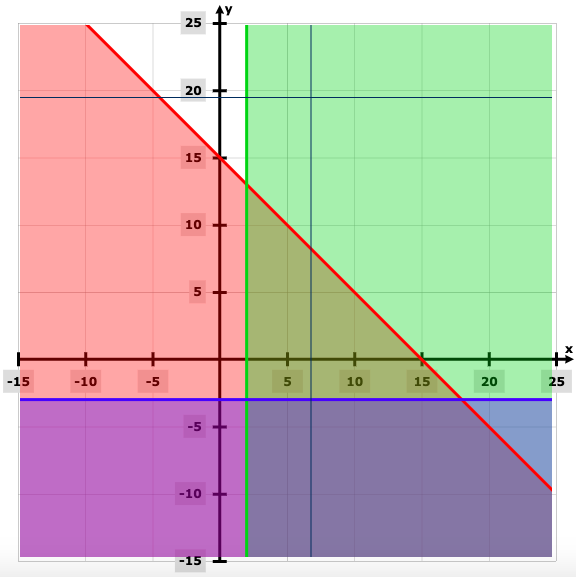(b)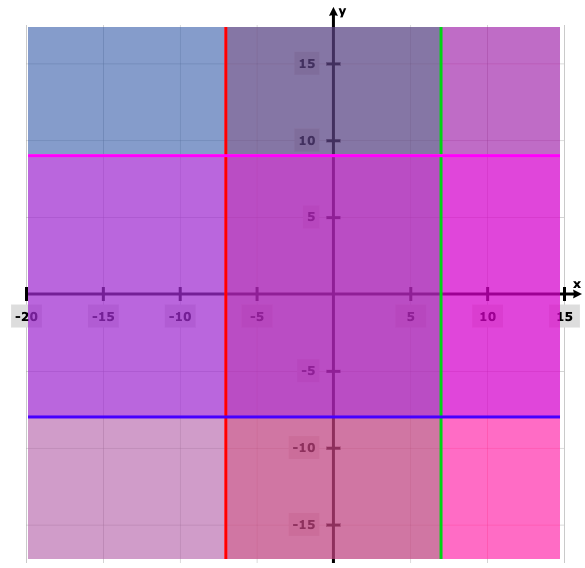Question 8: Answer any ONE question: [3 x 1 = 3]

[a] If x / y = (a + 2) / (a – 2), then show that (x2 – y2) / (x2 + y2) = 4a / a2 + 4.

[b] If bcx = cay = abz, then prove that (ax + by) / (a2 + b2) = (by + cz) / (b2 + c2).

Solution:

[a] x / y = (a + 2) / (a – 2)

(x2 / y2) = (a2 + 4a + 4) / (a2 – 4a + 4)

(x2 / y2) + 1 = [(a2 + 4a + 4) / (a2 – 4a + 4)] + 1

(x2 + y2) / y2 = 2a2 + 8 / (a2 – 4a + 4) —- (1)

Similarly

(x2 – y2) / y2 = 8a / (a2 – 4a + 4) —- (2)

Divide (2) and (1)

(x2 – y2) / (x2 + y2) = 8a / 2a2 + 8

(x2 – y2) / (x2 + y2) = 4a / a2 + 4

[b] Since a, b, c are not zero, the given constraint can be rewritten:

bcx = acy => bx = ay

acy = abz => cy = bz

abz = bcx => az = cx

Therefore

ab ( bx – ay ) + bc ( cy – bz ) + ca ( cx – az ) = 0

=> ab²x + bc²y + ac²x = a²by + b²cz + a²cz

=> ab²x + bc²y + ac²x + b³y = a²by + b²cz + a²cz + b³y

=> ( ax + by ) ( b² + c² ) = ( by + cz ) ( a² + b² )

=> ( ax + by ) / ( a² + b² ) = ( by + cz ) / ( b² + c² )

Question 9: Answer any ONE question: [3 x 1 = 3]

[a] If (x3 – (1 / y3)) ∝ (x3 + (1 / y3)) then show that x ∝ (1 / y).

[b] Volume of a cone is in joint variation with its square of the radius of the base and heights. The ratio of radii of the base of two cones is 3:4 and the ratio of their height is 6:5. Find the ratio of their volumes.

Solution:

[a] (x3 – (1 / y3)) ∝ (x3 + (1 / y3))

[(x3y3 – 1) / y3 ] / [(x3y3 + 1) / y3] = k

(x3y3 – 1) / (x3y3 + 1) = k

Using componendo and dividendo,

(x3y3 – 1 + x3y3 + 1) / (x3y3 – 1 – x3y3 – 1) = (k + 1) / (k – 1)

2x3y3 / (-2) = (k + 1) / (k – 1)

(-x3y3) = (k + 1) / (k – 1)

x3y3 = (k + 1) / (1 – k)

x3y3 = k (say some constant)

x3 = k / y3

So, x ∝ 1 / y

[b] Let there be cone 1 and cone 2 respectively.

Let the r and R be the radii of the two right circular cones respectively.

Ratio of base radii = 3:5

Ratio of their heights = 6:5

The volume of cone = 1 / 3 πr²h

⇒ The volume of cone 1 / Volume of cone 2

⇒ (1 / 3 * πr²h) / (1 / 3 πR²h)

⇒ (1 / 3 * π * 3² * 6) / (1 / 3 * π * 5² * 5)

= 3² * 6 / 5² * 5

= 54 / 125

So, the ratio of their volumes is 54:125.

Question 10: Answer any ONE question: [3 x 1 = 3]

[a] Simplify: 3√7 / (√5 + √2) – 5√5 / (√2 + √7) + 2√2 / (√7 + √5)

[b] If x = 2 + √3, y = 2 – √3, then find the value of xy + 1 / xy.

Solution:

[a]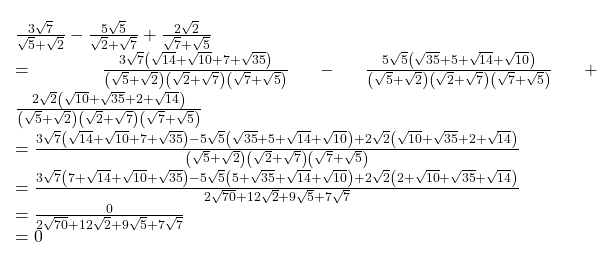[b] x = 2 + √3,

y = 2 – √3

xy + 1 / xy

= (2 + √3) * (2 – √3) + 1 / [2 + √3] * [2 – √3]

= [22 – 3 + 1] / [22 – 3]

= 2 / 4 – 3

= 2 / 1

= 2

Question 11: Answer any TWO questions: [5 x 2 = 10]

(a) Prove that the angle which an arc of a circle subtends at the centre is double the angle subtended by it at any point in the remaining part of the circle.

(b) Prove that a line segment drawn from the centre of a circle to bisect a chord which is not a diameter is at right angles to the chord.

(c) Prove that if a perpendicular is drawn from the vertex containing the right angle of a right-angled triangle on the hypotenuse the triangles on each side of the perpendicular are similar to each other.

Solution:

[a]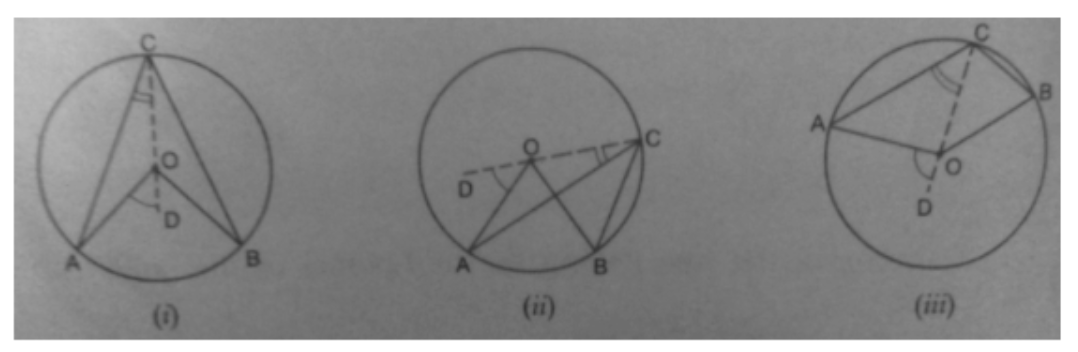Let there be a circle with a centre O. Arc AB intends AOB at the centre and ACB at any point C on the remaining part of the circle.

Construction: Join CO and produce it to any point D.

Proof:

OA = OC [radii of same circle ]

∠OAC = ∠ACO[angles opp to equal side’s of a triangle are equal]

∠AOD = ∠OAC + ∠ACO [exterior angles = sum of equal opposite angles]

∠AOD = 2(∠ACO)————-(1) [∠OAC = ∠ACO]

Similarly, ∠DOB = 2(∠OCB) ————-(2)

In fig (i) and (iii)

∠AOD + ∠ DOB = 2(∠ACO) + 2(∠OCB)

∠AOD + ∠ DOB = 2(∠ACO + ∠OCB)

∠AOB = 2(∠ACB)

In fig (ii)

subtracting (1) from (2)

∠DOB – ∠DOA = 2(∠OCB – ∠ACO)

∠AOB = 2(∠ACB)

In all the cases, ∠AOB = 2(∠ACB)

[b]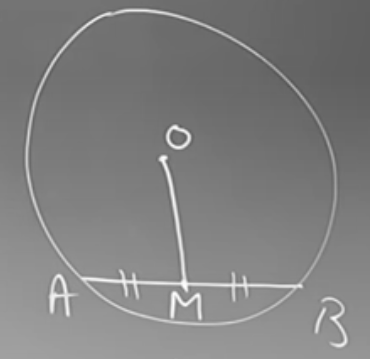Given O is the centre of the circle.

AB is the chord.

OM bisects AB at M.

AM = BM

Proof:

OM ⟂ AB

Join OA and OB

Consider △OAM and △OBM

AM = BM [M is the midpoint of AB]

OM = OM [common]

OA = OB [radii of the same circle]

So, △OAM ⩭ △OBM

∠OMA = ∠OMP [CACT]

∠OMA + ∠OMB = 180o [AB is a straight line]

∠OMA = ∠OMB = 90o

[c]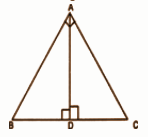Given a right angle triangle, right-angled at A.

AD is the perpendicular drawn to the hypotenuse BC from vertex A.

To Prove:

(i) ∆BDA ~ ∆BAC

Proof: In ∆BDA and ∆BAC:

∠B = ∠B [common]

Therefore, by using AA similar condition

∆BDA ~ ∆BAC …(i)

Now, in ∆ADC and ∆BAC, we have

∠C = ∠C [common]

Therefore, by using AA similar condition

Comparing (i) and (ii), we get

Question 12: Answer any ONE question: [3 x 1 = 3]

(a) There are two concentric circles such that two chords AB and AC of greater circle touch the smaller circle at P and Q respectively. Prove that PQ = (1 / 2)BC.

(b) ABCD is a rectangle and O is any point within it. Prove that OA2 + OC2 = OB2 + OD2.

Solution:

[a] Given: O is the centre of two concentric circles.

To prove: PQ = (1 / 2) BC

Construction: Join OP & OQ.

PROOF: The perpendicular from the centre to the chord bisects the chord.

AB = 2AP & AC = 2AQ

AB / AC = AP / AQ

∆APQ is similar to ∆ABC

AP / AB = PQ / BC

1 / 2 = PQ / BC

(1 / 2)BC = PQ

[b]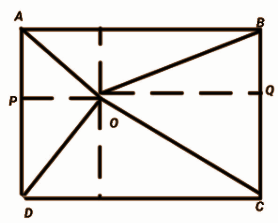Draw PQ ║AB ║CD as shown in the figure.

ABCD is a rectangle, it means ABPQ and PQDC are also rectangles.

For ABPQ,

AP = BQ [opposite sides are equal ]

For PQDC

PD = QC [opposite sides are equal]

For ∆OPD,

OD² = OP² + PD² ——(1)

For ∆OQB,

OB² = OQ² + BQ² ——–(2)

OB² + OD² = (OP² + PD²)+ (OQ² + BQ²) = (OP² + CQ²) + (OQ² + AP²)

In the figure, ∆OPA and ∆OQC are also right-angled triangles.

For, ∆OCQ ⇒ OQ² + CQ² = OC²

For, ∆OPA ⇒ OP² + AP² = OA² , put it above

Now,

OB² + OD² = OC² + OA²

Question 13: Answer any ONE question: [5 x 1 = 5]

(a) Draw a circumcircle of a triangle. (Only traces of construction are required)

(b) Find geometrically the value of √21. (Only traces of construction are required)

Solution:

1. Find the bisector of one of the triangle sides.

2. Repeat for another side.

3. Repeat for the third side. Three bisectors exist which intersect at a single point.

4. The point where these two perpendiculars intersect is the triangle’s circumcenter, the centre of the circle we desire.

4. Place the compasses’ point on the intersection of the perpendiculars and set the compasses’ width to one of the points A, B or C. Draw a circle that will pass through all three.

5. The circle drawn is the triangle’s circumcircle, the only circle that will pass through all three of its vertices.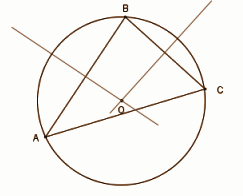[b]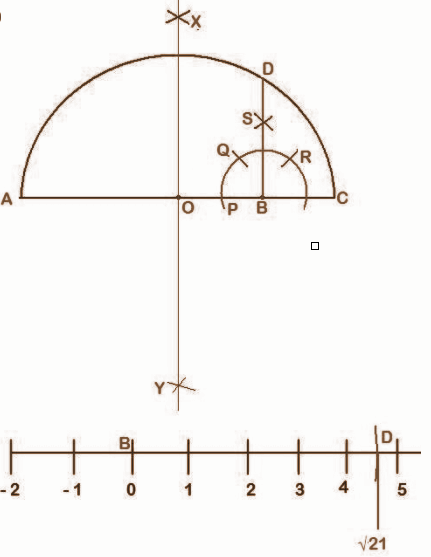Step 1: Draw a line AB = 21 units.

Step 2: Now extend line AB, as BC = 1 unit.

Step 3: Take radius as more than half of AC and with centres, A and C. Draw two arcs from both points on both sides of line AC, these arcs intersect at X and Y.

Step 4: Join XY. Line XY intersects line AC at O. Take radius OA = OC and draw a semicircle.

Step 5: Take any radius (less than BC) and centre B, draw a semicircle that intersects line AC at P. Now with the same radius and centre P, draw an arc that intersects our semicircle at Q. With same radius and centre Q, draw an arc that intersects our semicircle at R. With same radius and centre Q and R, draw these arcs that intersect at S.

Step 6: Join BS and extend that line intersect to the main semicircle at D.

Step 7: Now on taking point B as the origin and with a radius of BD, draw an arc that intersects our number line at D.

Question 14: Answer any ONE question: [3 x 1 = 3]

(a) A hemisphere and a cone are on equal bases and their heights are also equal. Find the ratio of the area of their curved surfaces.

(b) The base of a right prism of height 10 cm is a rectangle whose length and breadth are 5 cm and 3 cm, respectively. Find the volume of the prism.

Solution:

[a] Base of a cone and hemisphere are equal then their radius is also equal.

Radius and heights of the hemisphere and cone are the same.

Let the radius of the hemisphere = radius of the cone = r

Height of the cone = radius of the hemisphere = r

Slant height of a cone, l = √r² + h²

l = √r² + r²

l = √2r²

l = r√2 ………….(1)

The curved surface area of the hemisphere (S1) / Curved surface area of the cone (S2) = 2πr² /πrl

S1 / S2 = 2πr² / πrl

S1 / S2 = 2r / l

S1 / S2 = 2r / r√2 [From equation 1]

S1 / S2 = 2 / √2

S1 / S2 = (2 × √2) / (√2 × √2) [On rationalising the denominator]

S1 / S2 = 2√2 / 2

S1 / S2 = √2 / 1

S1 : S2 = √2 : 1

The ratio of the curved surface area of the hemisphere and cone = √2:1.

[b] Area of base = L * B

= 5 * 3

= 15cm2

The volume of the prism = base area * height

= 15 * 10

= 150 cm3

Question 15: Answer any ONE question: [4 x 1 = 4]

(a) 77 square metre tarpaulin needed to make a right circular conical tent. If the slant height of the tent is 7 m, find the area of the base of the tent.

(b) By melting two solid spheres of radii 1 cm and 6 cm, a hollow sphere of thickness 1 cm is made. Find the outer curved surface area of the new sphere.

Solution:

[a] Structure of a tent is like a right circular cone.

Tarpaulin required to make tent = curved surface area of the cone

Let, the base radius of the tent = ‘r’ m

Slant height = 7 m

⇒ π × r × 7 = 77

⇒ 22 / 7 × r = 11

⇒ r = 7 / 2 = 3.5

∴ Base radius of the tent = 3.5 m

∴ The base area of the tent = πr2

= 22 / 7 × 3.52

= 38.5 sq. m

[b] The volume of sphere 1 = (4 / 3) (22 / 7) * 13 = (88 / 21) * 1 cc

The volume of sphere 2 = (4 / 3) (22 / 7) * 63 = (88 / 21) * 216 cc.

The combined volume of the two spheres = (88 / 21) * 217 cc.

The hollow sphere has an outer radius of x and the internal radius = (x – 1).

Volume of the hollow sphere

= (4 / 3) (22 / 7) [x3 – (x – 1)3]

= (88 / 21) [x3 – (x – 1)3]

= (88 / 21) * 217

[x3 – (x – 1)3] = 217

x3 – (x3 – 3x2 + 3x – 1) = 217

x3 – x3 + 3x2 – 3x + 1 = 217

3x2 – 3x – 216 = 0

x2 – x – 72 = 0

(x – 9) (x + 8) = 0

x = 9 as -8 as negative value cannot be taken.

So the hollow sphere has a surface area of 4 * 𝛑 * 92

= 1018.29 sq cm.

Question 16: Answer any TWO questions: [3 x 2 = 6]

(a) A rotating ray revolves in the anticlockwise direction and makes two complete revolutions from its initial position and moves further to trace an angle of 30o. What are the sexagesimal and circular measures of the angle with reference to trigonometrical measure?

(b) If β and α are complementary angles to each other, then find the value of (1 – sin2α) (1 – cos2α) (1 + cot2β) (1 + tan2β).

(c) Prove that √1 + cos 30o / 1 – cos 30o = sec 60o + tan 60o.

(d) If cos 52o = x / √x2 + y2, then find the value of tan 38o.

Solution:

[a] One complete revolution = 360°.

So in two complete revolutions, it makes an angle of 360° × 2 = 720°.

30° is traced by moving further.

The magnitude of the angle formed is (720° + 30°)

= 750°.

= 750 π / 180

= 25 π / 6

[b] (1 – sin2α) (1 – cos2α) (1 + cot2β) (1 + tan2β)

= cos2 α * sin2 α * cosec2 β * sec2 β

α + β = 90o

β = 90 − α

cos β = cos (90 − α)

cos β = sin α

β = 90 − α

sin β = sin (90 − α)

sin β = cosα

= cos α * cos α * sin α * sin α * (1 / sin2 β) * (1 / cos2 β)

= cos α * cos α * sin α * sin α * (1 / sin α * sin α) * (1 / cos α * cos α)

= 1

[c] √1 + cos 30o / 1 – cos 30o

= (√1 + cos 30o / 1 – cos 30o) * (√1 + cos 30o / 1 + cos 30o)

= (√(1 + cos2 30o)2 / (1 – cos2 30o)

= (√1 + cos 30o / sin 30o

= cosec 30o + cot 30o

[d] cos 52o = x / √x2 + y2

tan 38o = ?

cos (90o – 52o) = x / √x2 + y2

sin α = P / H

By the Pythagoras theorem,

H2 = P2 + B2

B = √H2 – P2

= √x2 + y2 – x2

= y

tan 380 = P / B = x / y

Question 17: Answer any ONE question: [5 x 1 = 5]

(a) From a quay of a river, 600 metres wide, two boats start in two different directions to reach the opposite side of the river. The first boat moves to make an angle of 30o with the bank and the second boat moves to make an angle of 90o with the direction of the first board. What will be the distance between the two boats when both of them reach the other side?

(b) The height of the two towers is h1 and h2 respectively. If the angle of elevation of the top of the first tower from the foot of the second tower is 60o, and the angle of elevation of the top of the second tower from the foot of the first tower is 45o, then show that h12 = 3h22.

Solution:

[a] tan 30° = width of river/distance between the two boats when both of them reach the other side

=> 1 / √3 = 600 / distance between the two boats when both of them reach the other side

=> distance between the two boats when both of them reach the other side = 600√3 m

= 1039.2 m

1039.2 m will be the distance between the two boats when both of them reach the other side.

[b]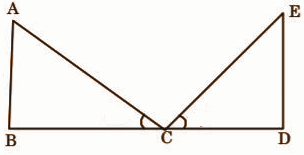tan 60 = AB / BC

√3 = H1 / x

x = H1 / √3

tan 45 = DE / DC

1 = H2 / x

x = H2

H1 / √3 = H2

Squaring both sides,

H12 = 3H22

Question 18: [Alternative Questions for Sightless Candidates]

Find the coordinates of three points lying in the solution region satisfying the inequalities: 4x + 3y ≤ 12; x ≥ 0, y ≥ 0. [4M]

Solution: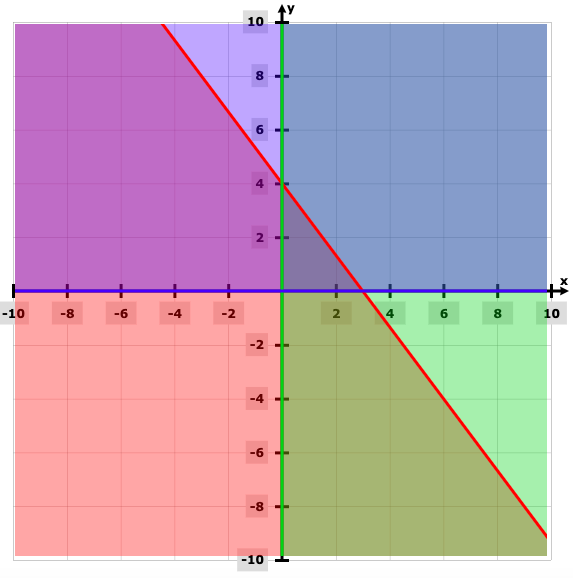(2, 1), (0, 4) and (3, 0) are the coordinates of three points lying in the solution region satisfying the given inequalities.

Question 19: Answer any ONE question: [5 x 1 = 5]

(a) Describing the procedure of constructing the circumcircle of a triangle

whose length of three sides are given?

(b) Describe the procedure of drawing a tangent at a point on a circle.

Solution:

[a]

1. Find the bisector of one of the triangle sides.

2. Repeat for another side.

3. Repeat for the third side. three bisectors exist which intersect at a single point.

4. The point where these two perpendiculars intersect is the triangle’s circumcenter, the centre of the circle we desire.

4. Place the compasses’ point on the intersection of the perpendiculars and set the compasses’ width to one of the points A, B or C. Draw a circle that will pass through all three.

5. The circle drawn is the triangle’s circumcircle, the only circle that will pass through all three of its vertices.[b] Construction of a tangent to a circle

To draw a tangent to a circle at a point on the circle. (Refer fig.)

Step 1: Draw a circle with the required radius with centre O.

Step 2: Join the centre of the circle O and any point P on the circle. OP is the radius of the circle.

Step 3: Draw a line perpendicular to radius OP through point P. This line will be a tangent to the circle at P.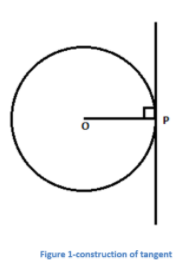(a) 75% of A = 50% of B; find A:B. 

(b) Find the mean proportion of 6a3b and 24ab3 

(c) If tan (θ + 15o) = √3, find sin θ. 

(d) Find the value of 𝛑 / 12 radians in degree. 

(e) If the length of each side of a rhombus is 5 cm and the length of one diagonal is 8 cm, find the length of the other diagonal. 

(f) How many solid right circular cones can be formed by melting a solid cylinder having equal radius and height? 

Solution:

[a] 75% of A = 50% of B.

[75 / 100] * A = [50 / 100] * B

75A / 100 = 50B / 100

3A / 4 = B / 2

6A = 4B

6 / 4 = A / B

3 / 2 = A / B

A:B = 3:2

[b] 6a3b and 24ab3

Mean proportion = (6a3b * 24ab3)½

= (144 a4b4)½

= 12a2b2

[c] tan (θ + 15o) = √3

(θ + 15o) = tan-1 (√3)

θ + 15o = 60

θ = 60o – 15o

θ = 45o

= x * (180 / 𝛑)

= [𝛑 / 12] * (180 / 𝛑)

= 180o / 12

= 15o

[e] The diagonals of a rhombus intersect at the right angle.

⇒ Half of each diagonal and the length of the side will form a right angle triangle.

Diagonal = 8 cm

1/2 the diagonal = 8 ÷ 2 = 4 cm

a² + b² = c²

a² + 4² = 5²

a² = 5² – 4²

a² = 9

a = √9

a = 3 cm

1 / 2 the diagonal = 3 cm

Diagonal = 3 x 2 = 6 cm

The length of the other diagonal is 6 cm.

[f] Two solid right circular cones.